# Modelling Time-To-Event Data in Chickens – Towards AI#### Author(s): Dr. Marc Jacobs

Originally published on Towards AI the World’s Leading AI and Technology News and Media Company. If you are building an AI-related product or service, we invite you to consider becoming an AI sponsor. At Towards AI, we help scale AI and technology startups. Let us help you unleash your technology to the masses.

#### Combining mortality and temperature data for an exciting endeavor

This post will NOT be about Kaplan-Meier or Cox Regression, but about modeling time-to-event data via the Binary, Poisson, or Negative-Binomial way. If you are interested in any of these topics, in different examples, just click on the links attached to them.

What I will add in this post is something additional that can be a real pain in the ass — I am modeling the data coming from a time-series dataset. This means an almost full year of data, coming from laying hens, in which hens died, and when you have almost 20k hens that happen. Even if you do not wish it to be. Now, a well-known catalyst of mortality, or at the very least production loss, is temperature. Despite well-controlled measures to ensure barn temperature is stable, there ARE fluctuations. So, what we will have, as a dataset, are event data (discrete and ordinal) and continuous data. The temp data we have on an hourly basis, and the event data are daily.

Let’s see where we end up, but I already know this will not be easy. Love it!

First, the libraries. Always, the libraries!

rm(list = ls())
options(“scipen”=100, “digits”=10)
#### LIBRARIES ####
library(DataExplorer)
library(tidyverse)
library(gridExtra)
library(grid)
library(AzureStor)
library(skimr)
library(lubridate)
library(ggplot2)
pacman::p_load(tidyverse, magrittr) # data wrangling packages
timetk, modeltime.gluonts, tidymodels, modeltime.ensemble, modeltime.resample) # time series model packages
theme_set(hrbrthemes::theme_ipsum()) # set default themes

Then, I will load the data from blob storage on Azure into R and take a good look at it.

folder<-setwd(getwd())
name=”/DATA.csv”
dest = paste0(folder,name),
overwrite = TRUE,
key = “xxxxxxxxxxxxxxxxxxxxxxxxxxxxxxxxxxx”)
skimr::skim(day)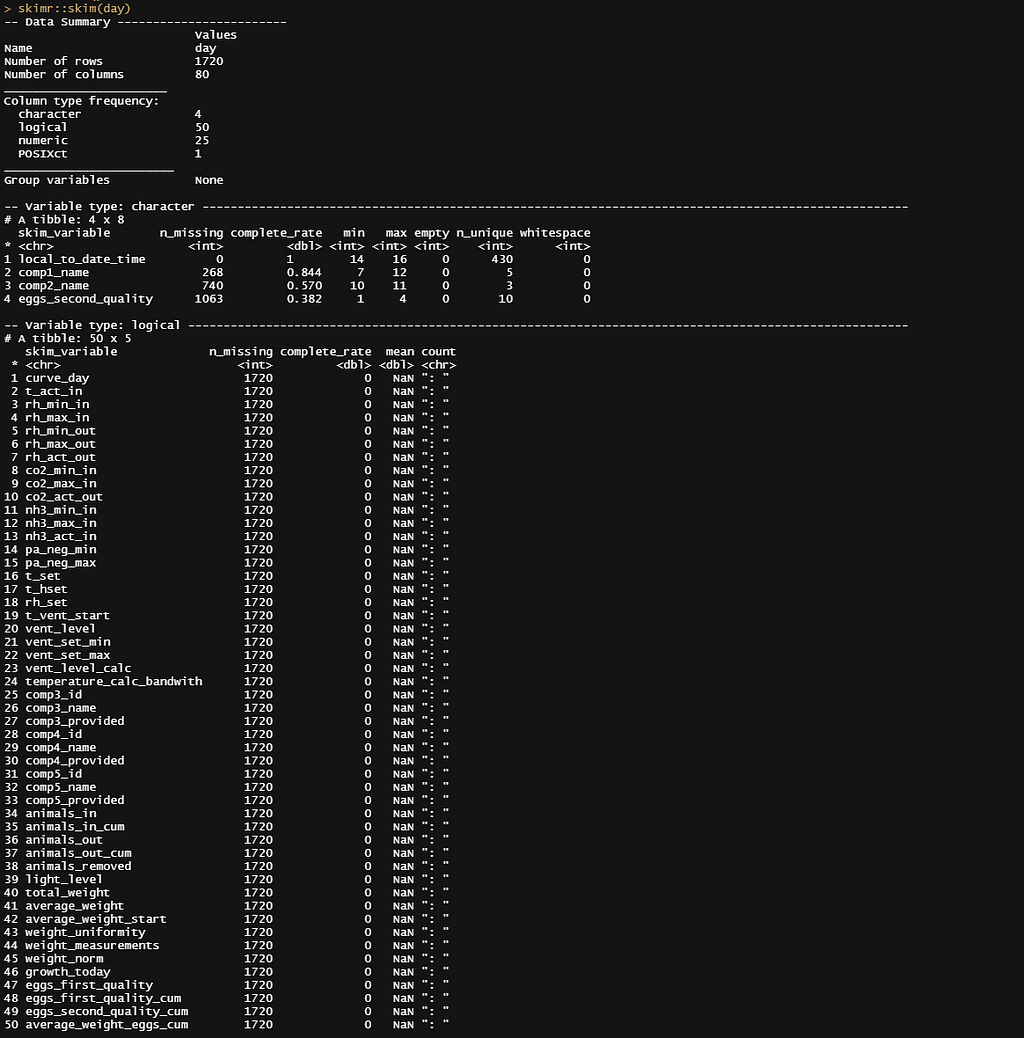With 80 columns, the data is decent. Plenty of stuff you can do with it, but I will be focusing on a majority of perhaps 10 variables.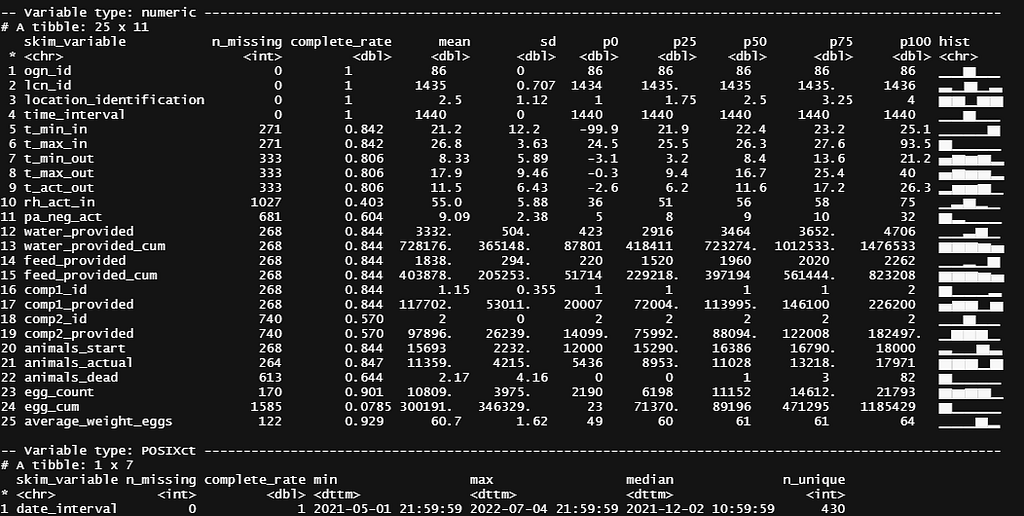And the numeric data. It is here you can see temperature variables, such as t_min_in or t_act_out. We will dive deeper into those a bit later on.

To get some grip on the data, let’s start plotting. Now, the fun part about this dataset is that you easily plot curves resembling survival curves, but the data is not set up in such a way that you can perform survival analysis on it.

day%>%
dplyr::filter(!lcn_id %in% c(‘1435’))%>%
ggplot(.,
aes(x=date_interval,
y=animals_actual,
colour=as.factor(lcn_id)))+
geom_line()+
facet_wrap(~lcn_id, scales=”free”)+
labs(x=”Time”,
y=”Animals”,
col=”Barn”)+
theme_bw()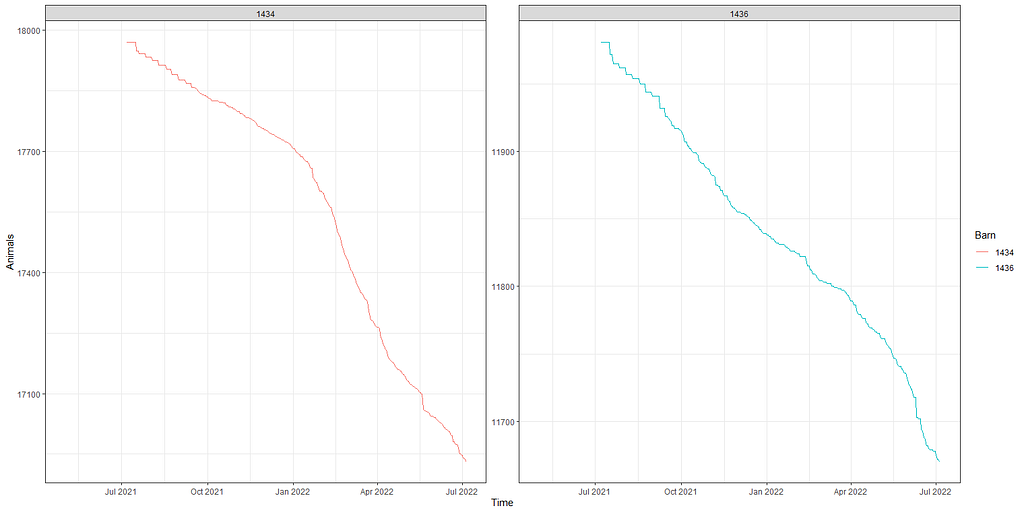A plot, showing for two barns, the actual animals per barn over time. The pictures mimic a survival plot, but the data is NOT in survival analysis mode. I will show later why that is not necessary — we won’t be doing survival analysis anyhow.

Above you saw the change in actual animals, but down below I will show you the actual deaths per time point. As you can see, the two barns differ in their mortality pattern.

day%>%
dplyr::group_by(lcn_id)%>%
dplyr::filter(!lcn_id %in% c(‘1435’))%>%
ggplot(.,
aes(x=date_interval,
fill=as.factor(lcn_id)))+
geom_bar()+
facet_wrap(~lcn_id, scales=”free”)+
labs(x=”Time”,
fill=”Barn”)+
theme_bw()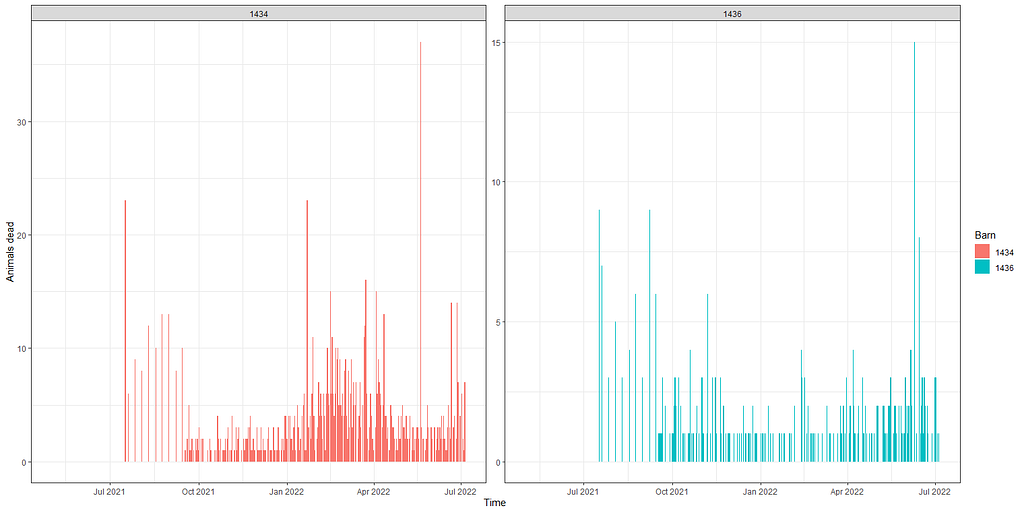day%>%
dplyr::group_by(lcn_id)%>%
dplyr::filter(!lcn_id %in% c(‘1435’))%>%
replace(is.na(.), 0)%>%
ggplot(.,
aes(x=date_interval,
y=as.factor(lcn_id),
fill=as.factor(event)))+
geom_tile()+
scale_fill_viridis_d(option=”cividis”)+
labs(x=”Time”,
y=”Barn”,
fill=”Event”)+
theme_bw()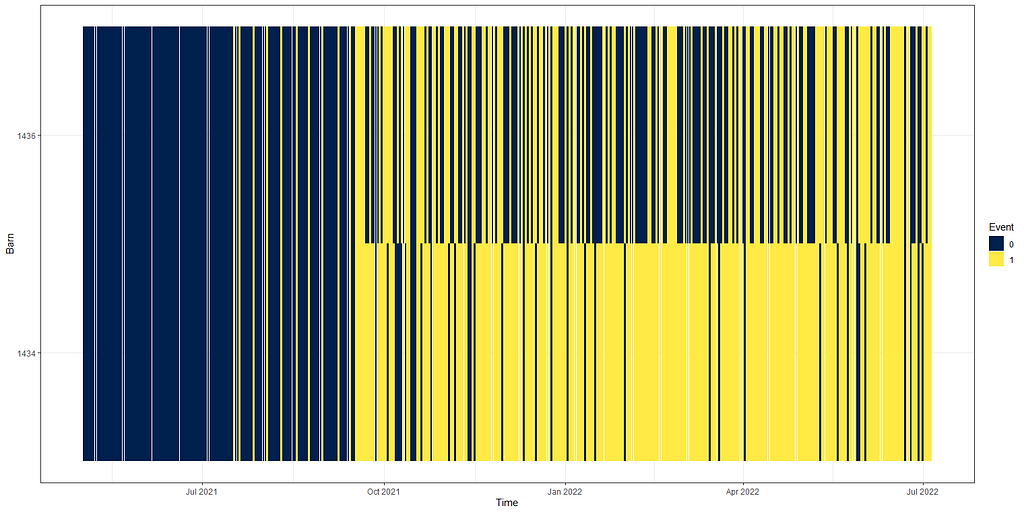The actual animals across time, however, are not only decimated by death. It can very well be that birds are moved or taken out for whatever reason. Let’s take a look at the curves when we only take death as subtraction.

day%>%
dplyr::filter(!lcn_id %in% c(‘1435’))%>%
dplyr::filter_at(vars(animals_start), all_vars(!is.na(.)))%>%
replace(is.na(.), 0)%>%
ggplot(.,
aes(x=date_interval))+
geom_line(aes(y=animals_actual), col=”red”)+
facet_wrap(~lcn_id, scales=”free”)+
labs(x=”Time”,
y=”Animals”)+
theme_bw()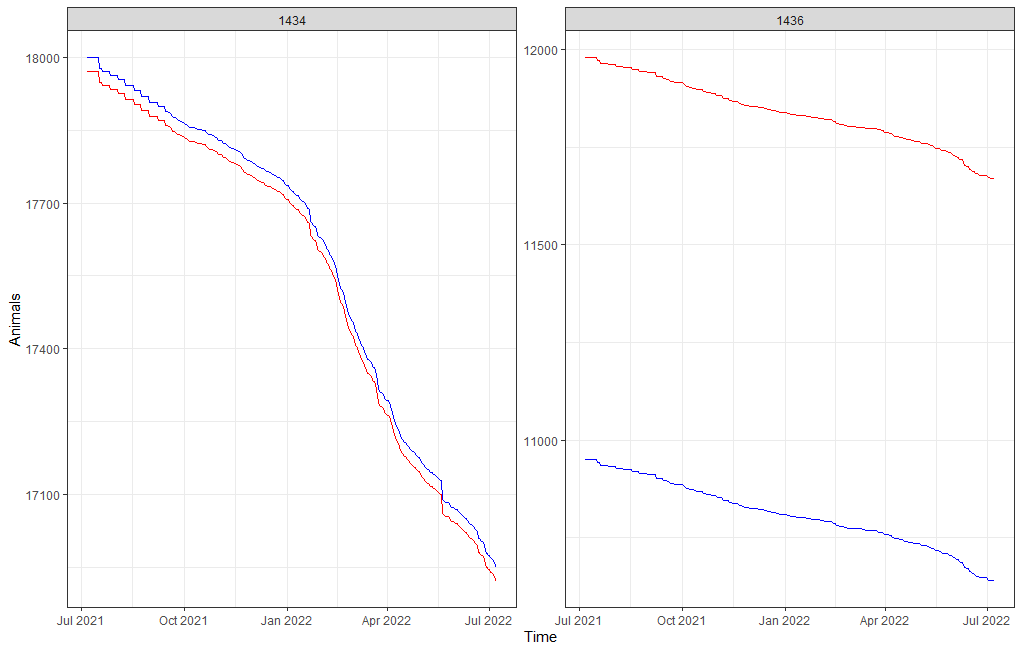Something went amiss, but below it straightens out. As you can see, the curve obtained by just looking at death is almost parallel to the overall actual animal curve. So nothing to worry about here.

day%>%
dplyr::filter(lcn_id == c(‘1436’))%>%
dplyr::filter_at(vars(animals_start), all_vars(!is.na(.)))%>%
replace(is.na(.), 0)%>%
ggplot(.,
aes(x=date_interval))+
geom_line(aes(y=animals_actual), col=”red”)+
facet_wrap(~lcn_id, scales=”free”)+
labs(x=”Time”,
y=”Animals”)+
theme_bw()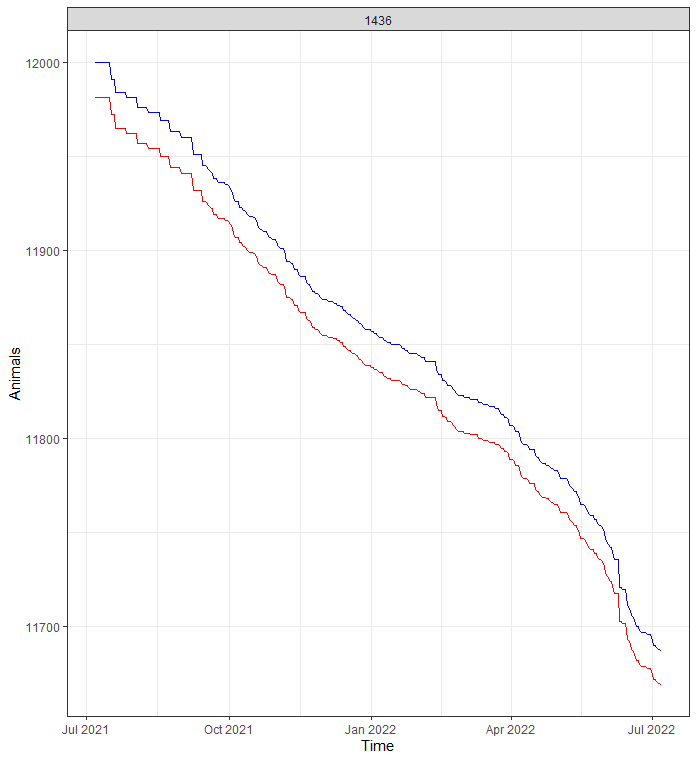Now, what will make this exercise downright dirty is that I will have to model event data over time but without using survival techniques. Potential options are the binary class or binomial class if I aggregate per week or month. The binary can be a real pain and the model can take ages to run, having to find patterns within the 0’s and 1’s. For those who have forgotten what a Binary or Bernoulli, distribution looks like: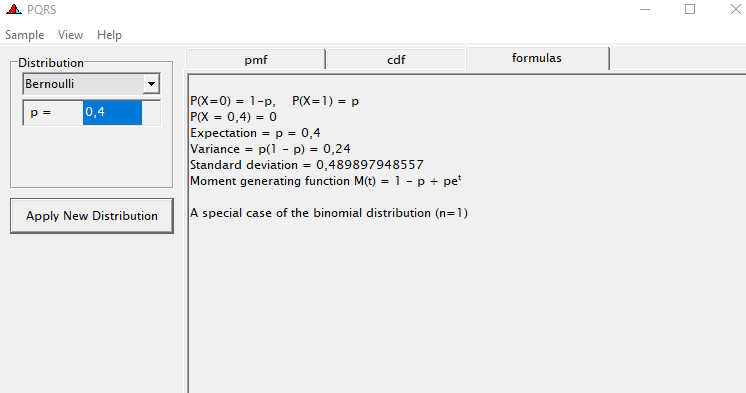In Bernoulli, all you have is a chance and that will determine everything. In the data, all you will see is a zero or a one, and those values are NOT connected like in a Binomial. There is NO variation to look for, except from what you see in EACH row and that is why it takes so long to run. I will show you later the agony it may cause.

Let’s get to the modeling part and run a binary model over time, and see how that goes. Nothing fancy.

day%>%
dplyr::filter(!lcn_id %in% c(‘1435’))%>%
dplyr::filter_at(vars(animals_start), all_vars(!is.na(.)))%>%
dplyr::mutate(day = lubridate::day(date_interval))%>%
replace(is.na(.), 0)%>%
glm(event~
day+as.factor(lcn_id),
data=.)%>%summary()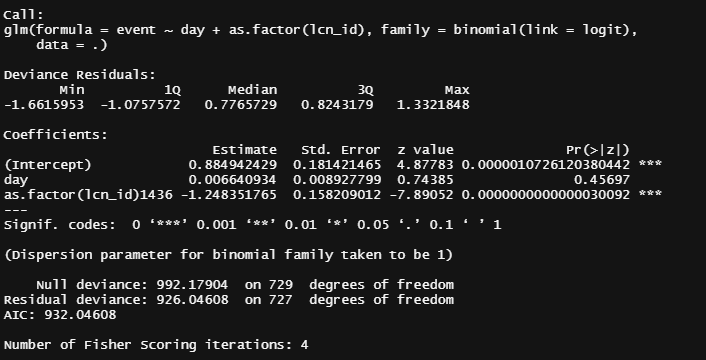The glm model trying to connect the probability of an event to the day and the location. The model is linear.

It is always best to plot what the model came up with, the numbers above do not really help I believe.

fit<-day%>%
dplyr::filter(!lcn_id %in% c(‘1435’))%>%
dplyr::filter_at(vars(animals_start), all_vars(!is.na(.)))%>%
dplyr::mutate(day = lubridate::day(date_interval))%>%
replace(is.na(.), 0)%>%
glm(event~
day+as.factor(lcn_id),
data=.)
plot(fit)
sjPlot::plot_model(fit, type=”pred”,
terms=c(“day [all])”, “lcn_id”))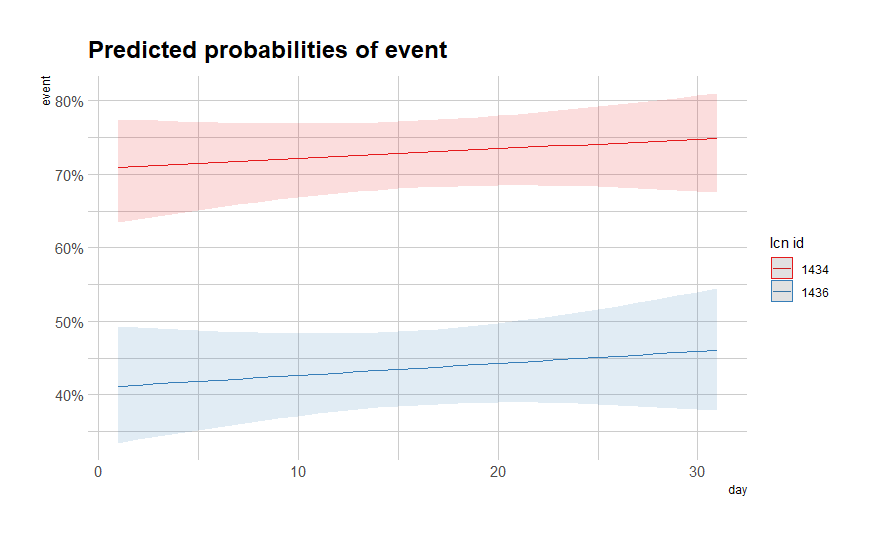We all know from the previous plots that the pattern is not linear. So what if we add a spline to it? Because we have quite a number of days included in the dataset, we can create a nice spline to the fifth order. Creating a heavier spline would probably require modeling via sine and/or cosine.

fit<-day%>%
dplyr::filter(!lcn_id %in% c(‘1435’))%>%
dplyr::filter_at(vars(animals_start), all_vars(!is.na(.)))%>%
dplyr::mutate(day = lubridate::day(date_interval))%>%
replace(is.na(.), 0)%>%
glm(event~
splines::ns(day,5)+as.factor(lcn_id),
data=.)
summary(fit)
sjPlot::plot_model(fit, type=”pred”,
terms=c(“day [all])”, “lcn_id”))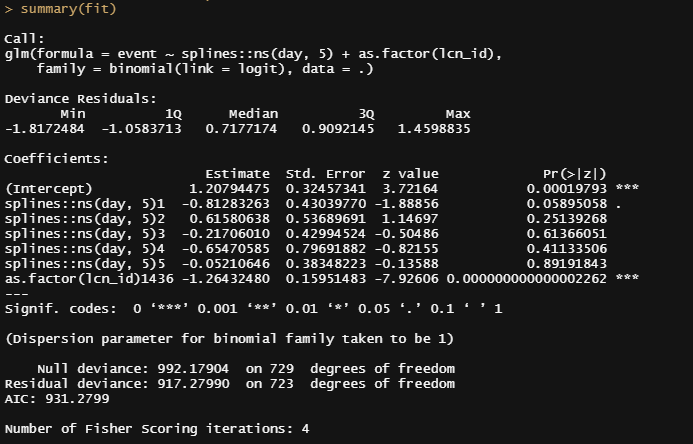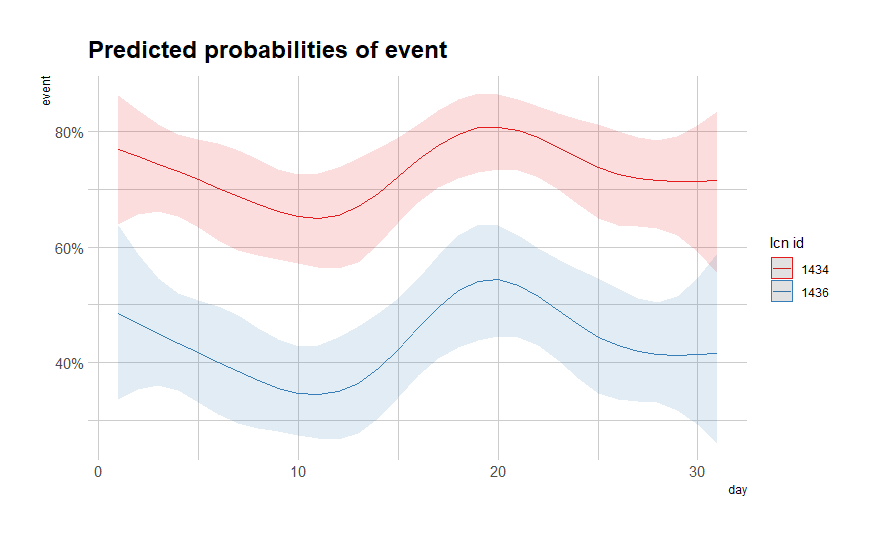Incorporating splines will make the analysis look like this. Now, what happened is that the model has aggregated all the data across the 31 days of a months, creating variance which should not be there. I want to model the entire 300+ days but the model is not to blame. The mistake is mine.

So, let’s model it a bit better, taking the month into account, and see what we end up with.

df_fit2<-fit2<-day%>%
dplyr::filter(!lcn_id %in% c(‘1435’))%>%
dplyr::filter_at(vars(animals_start), all_vars(!is.na(.)))%>%
dplyr::mutate(day = lubridate::day(date_interval),
month=lubridate::month(date_interval))%>%
dplyr::select(lcn_id, day, month, animals_dead, animals_start, animals_actual)%>%
replace(is.na(.), 0)%>%
dplyr::select(lcn_id, day, month, event)
pred<-plogis(predict(fit2, newdata=df_fit2))
plot(pred)
df_fit2_combined<-cbind(df_fit2, pred)
ggplot(df_fit2_combined,
aes(x=day, y=pred, col=as.factor(lcn_id)))+
geom_point(alpha=0.5)+
geom_line()+
facet_wrap(~month, scales=”free”)+
labs(x=”Day in month”, y=”Predicted Probability of Mortality”, col=”Location”)+
theme_bw()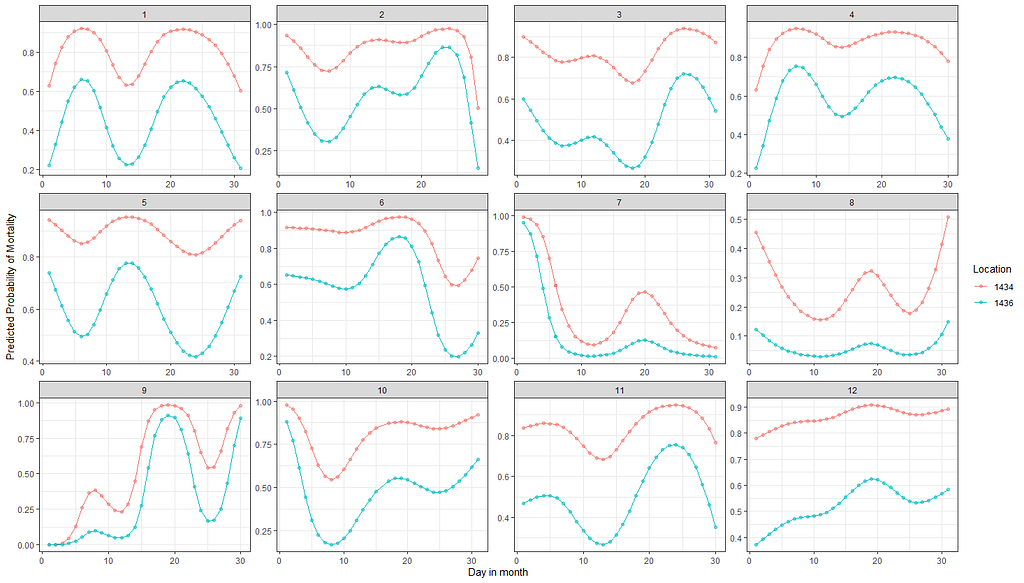You can see the curves differ per month, but the distance is always the same. That is because I did not include an interaction. Lets do that.
fit3<-glm(event~
splines::ns(day,5)*as.factor(month)*as.factor(lcn_id),
data=df_fit2)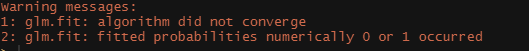And now we end with this, which makes perfect sense. I have included all possible interactions meaning that there is no error left. So, what to do?

We can specify in the model which interactions we want to have and so I chose to interact day*lcn_id and month*lcn_id. What I will get is not that much different from what I already had.

fit3<-glm(event~
splines::ns(day,5) + as.factor(month) + as.factor(lcn_id) +
splines::ns(day,5):as.factor(lcn_id) + as.factor(month):as.factor(lcn_id),
data=df_fit2)
summary(fit3)
pred_fit3<-plogis(predict(fit3, newdata=df_fit2))
plot(pred_fit3)
df_fit3_combined<-cbind(df_fit2, pred_fit3)
ggplot(df_fit3_combined,
aes(x=day, y=pred, col=as.factor(lcn_id)))+
geom_point(alpha=0.5)+
geom_line()+
facet_wrap(~month, scales=”free”)+
labs(x=”Day in month”, y=”Predicted Probability of Mortality”, col=”Location”)+
theme_bw()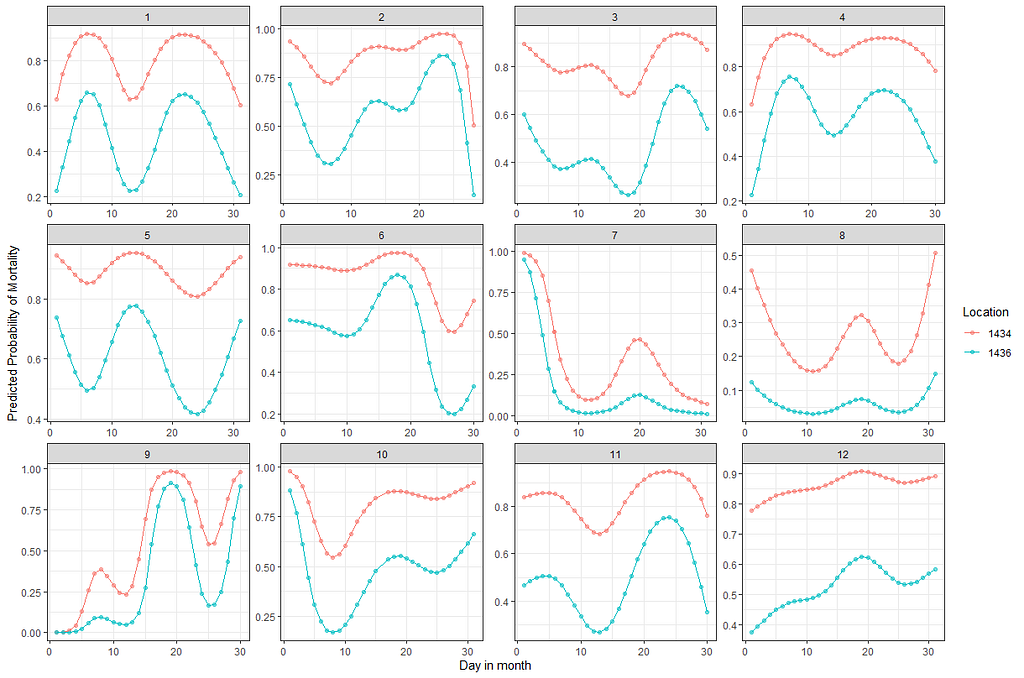Can’t say I see a difference.

Let’s compare the models we have up until now.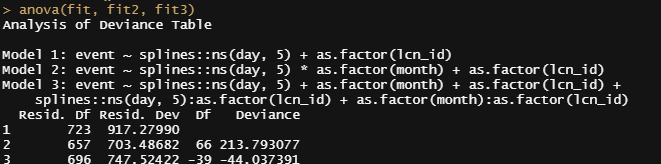Does not seem the last model is really worth the effort. But that was already somewhat clear from the graphs above. At least, they did not differ that much.

Then again, perhaps they are all really not that helpful, considering that more than one bird can die in a day and we did not take into account the fact that we start with 18k birds, to begin with. So the chance of dying is certainly not 80% and the fact that we will encounter dead birds over the course of time is a certainty (that is actually what the 80% stands for). Hence, the high probability, and time to move to the Binomial and Poisson distribution and calculate some ratios and rates.

fit<-day%>%
dplyr::filter(!lcn_id %in% c(‘1435’))%>%
dplyr::filter_at(vars(animals_start), all_vars(!is.na(.)))%>%
dplyr::mutate(day = lubridate::day(date_interval),
month=lubridate::month(date_interval))%>%
replace(is.na(.), 0)%>%
splines::ns(day,5)*as.factor(month)+as.factor(lcn_id),
data=.)
summary(fit)
sjPlot::plot_model(fit)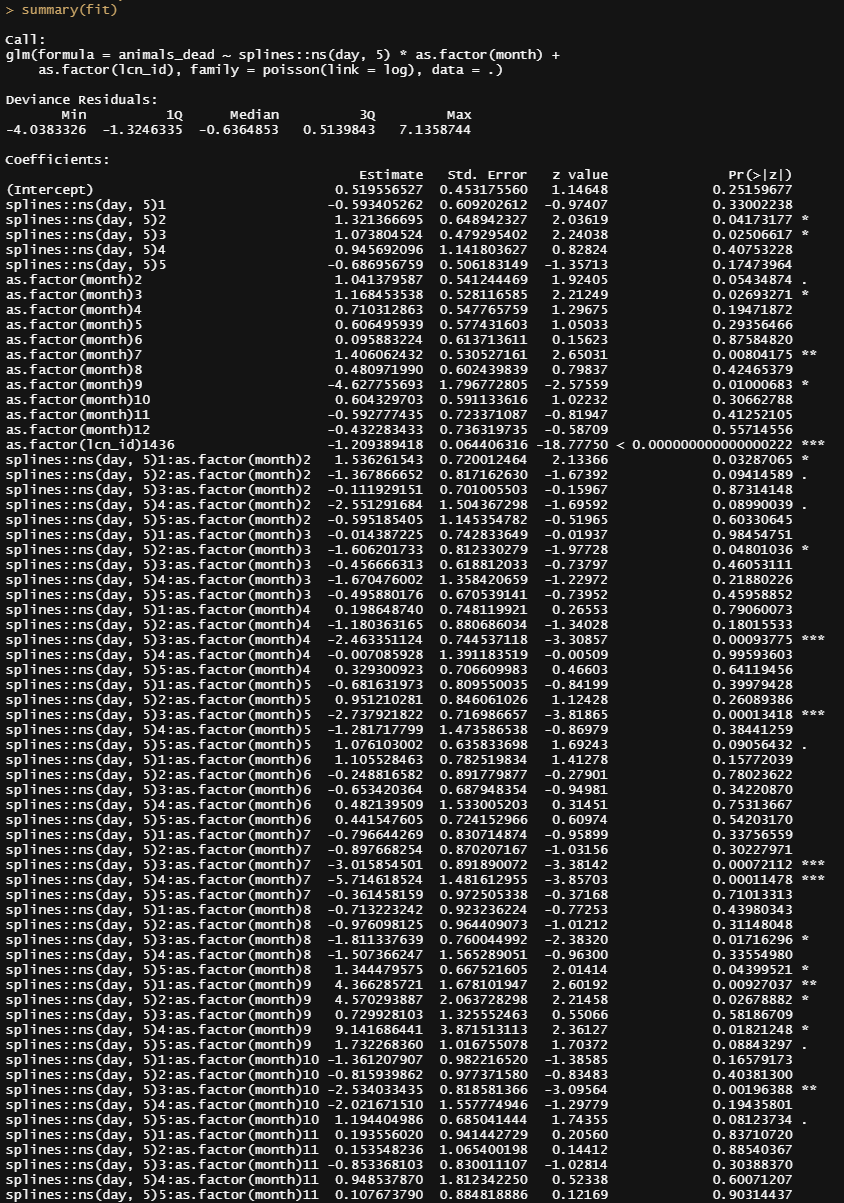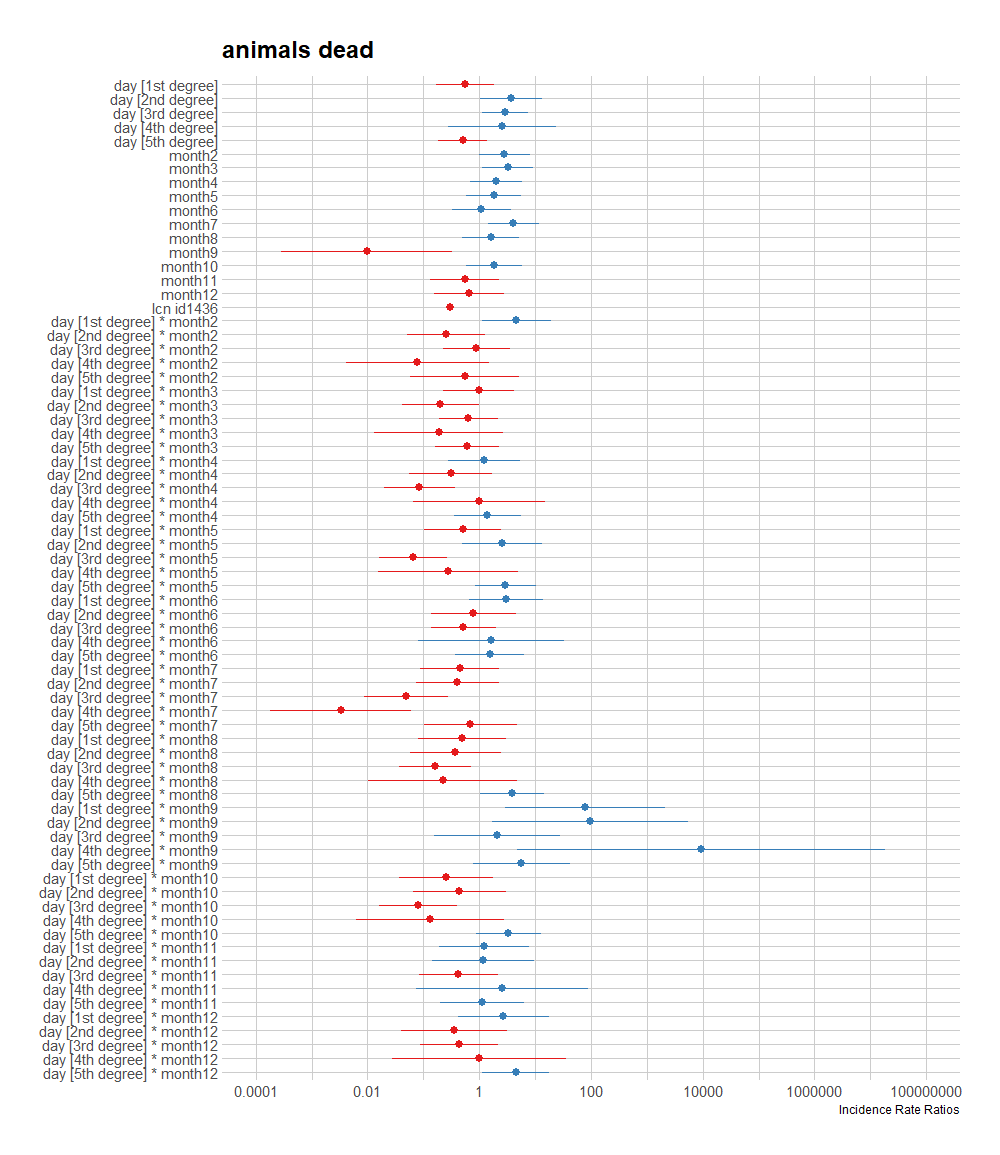Yes, with such a model and interactions you will get a load of parameters. Imagine creating priors for such a model when doing Bayesian calculations. Then again, that would not be too bad. Some parameters have insane incidence rate ratios which, at this point, is unclear if that is ‘real effect’ or an error due to variation issues. The large confidence intervals seem to hint at the latter, but I am not sure.

A plot coming from the sjPlot package is giving me issues if I want to plot everything, so all the parameters. What I can do, for now, is look at the predicted counts, average per month, and per location. There seems to be a pattern, but I am highly uncertain if that pattern is indeed any good.

sjPlot::plot_model(fit, type=”pred”,
terms=c(“month”, “lcn_id”))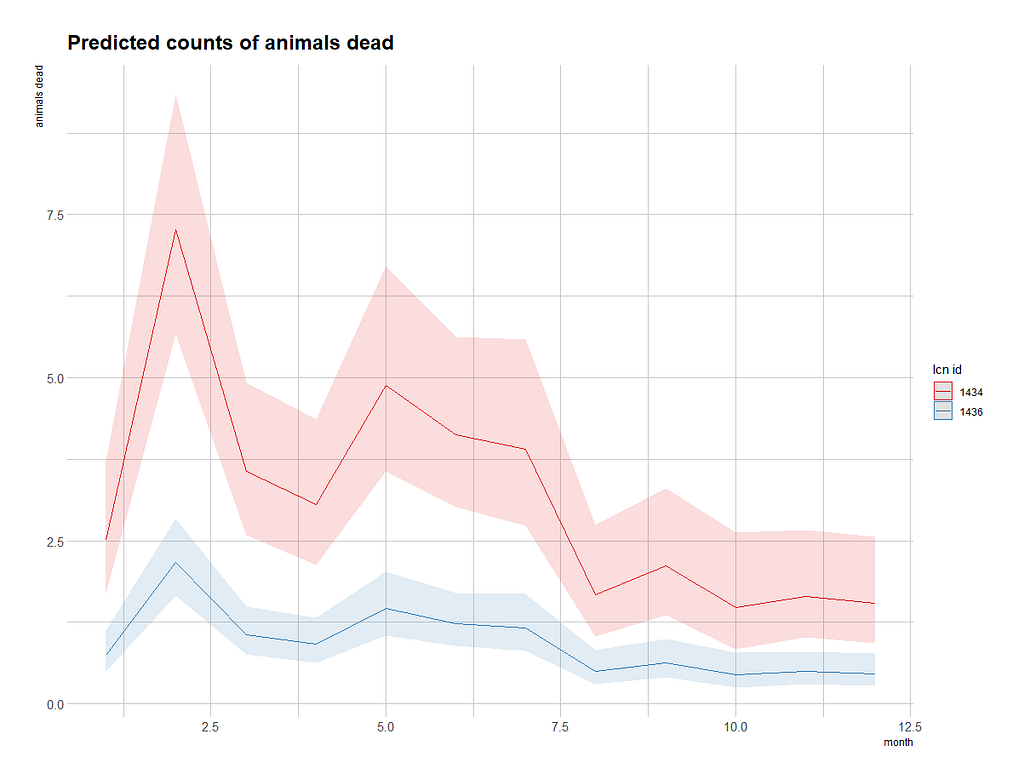To get a better view of the conditional effect, I am going to ask R to give me the predicted count which I will obtain by exponentiating the log value I receive from glm. This way I will be able to plot across all potential influencers counts and see if they make sense.

df_pois<-day%>%
dplyr::filter(!lcn_id %in% c(‘1435’))%>%
dplyr::filter_at(vars(animals_start), all_vars(!is.na(.)))%>%
dplyr::mutate(day = lubridate::day(date_interval),
month=lubridate::month(date_interval))%>%
replace(is.na(.), 0)
splines::ns(day,5)*as.factor(month)+as.factor(lcn_id),
data=df_pois)
pred_fit<-exp(predict(fit,newdata=df_pois))
df_fit_combined<-cbind(df_pois, pred_fit)
ggplot(df_fit_combined,
aes(x=day,
y=pred_fit,
col=as.factor(lcn_id)))+
geom_point(alpha=0.5)+
geom_line()+
facet_wrap(~month, scales=”free”)+
labs(x=”Day in month”,
y=”Predicted Counts of Mortality”,
col=”Location”)+
theme_bw()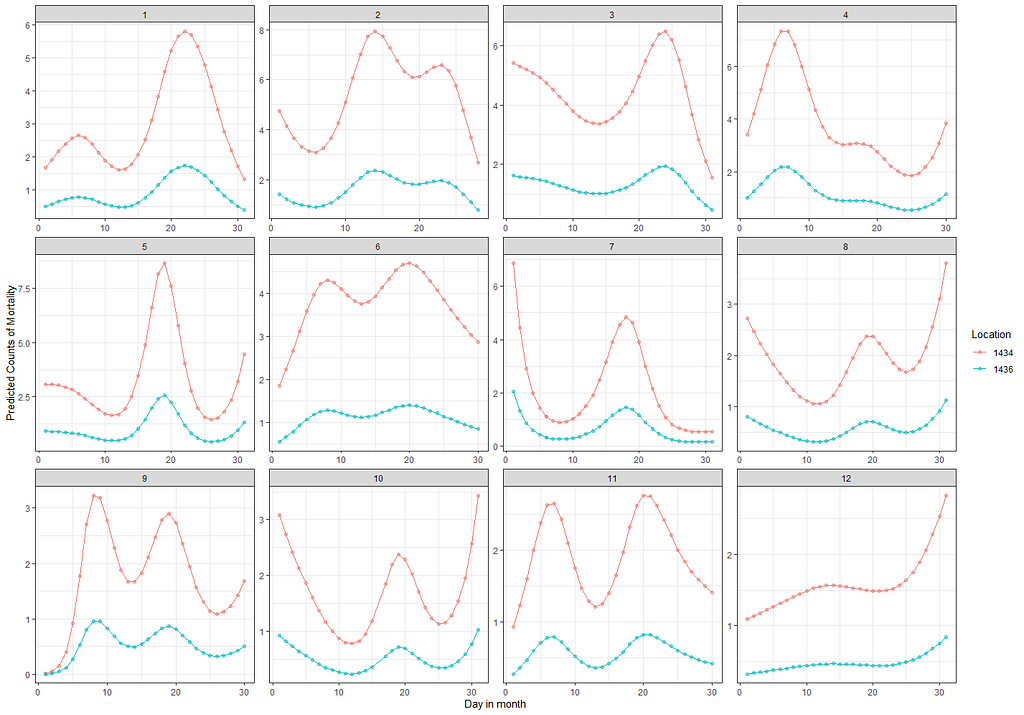Plots show predicted counts per location over time. If these predictions are any good I do not know yet but I will return to the topic later on.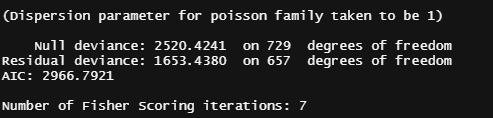Ah, the dispersion parameter. I talked about this one before and what I am seeing here friends IS overdispersion. And a lot of it. Meaning that the model is underestimating the actual variance which does not surprise me. Poisson models are inherently limited.

From the beginning, I mentioned that I want to connect the mortality numbers to temperature since heat stress is a known cause of the severe disruption. To protect from that barns have highly sensitive monitoring systems that control the temperature. We will see that later in the plots as well. Below, I will show you the minimum and maximum temp in the barn, and the actual temp outside.

day%>%
dplyr::filter(!lcn_id %in% c(‘1435’))%>%
dplyr::filter_at(vars(animals_start), all_vars(!is.na(.)))%>%
dplyr::select(lcn_id, date_interval, t_min_in, t_max_in, t_act_out)%>%
replace(is.na(.), 0)%>%
ggplot(.,
aes(x=date_interval))+
geom_line(aes(y=t_max_in), col=”red”)+
geom_line(aes(y=t_min_in), col=”blue”)+
geom_line(aes(y=t_act_out), col=”black”)+
facet_wrap(~lcn_id, scales=”free”)+
labs(x=”Time”,
y=”Temperature in the barn”)+
theme_bw()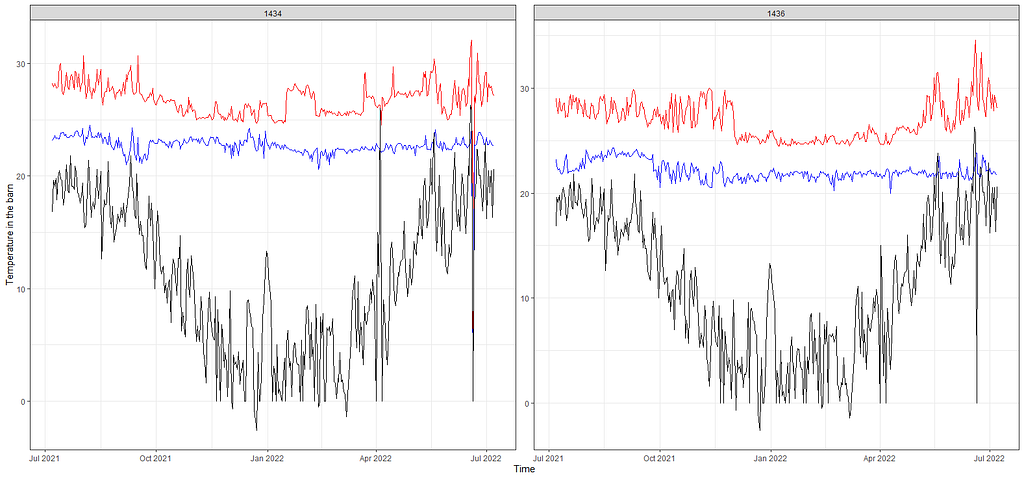The data shows fluctuations, as can be expected. Now, the real fun is to see if we can connect such data with the event data that we have.

Keep in mind that time-series data, such as temperature data, have components in them that are not directly visible. It could very well be that I need to disentangle these components, for instance, some form of seasonality or cyclical pattern, to connect the temperature data to the event data. Also, we have the data on a daily level. I do have access to hourly data, but that would add more complexity to the analysis. Let’s keep it in the back of our minds though.

First, I want to visualize the data in a more easy-to-read way and heatmaps do the trick.

day%>%
dplyr::filter(!lcn_id %in% c(‘1435’))%>%
dplyr::filter_at(vars(animals_start), all_vars(!is.na(.)))%>%
dplyr::select(lcn_id, date_interval, t_min_in, t_max_in, t_act_out)%>%
replace(is.na(.), 0)%>%
reshape2::melt(id.vars=c(“lcn_id”, “date_interval”))%>%
ggplot(.,
aes(x=date_interval,
y=variable,
fill=value))+
geom_tile()+
scale_fill_viridis_c(option=”turbo”)+
facet_wrap(~lcn_id, scales=”free”)+
labs(x=”Time”,
y=”Temperatures”)+
theme_bw()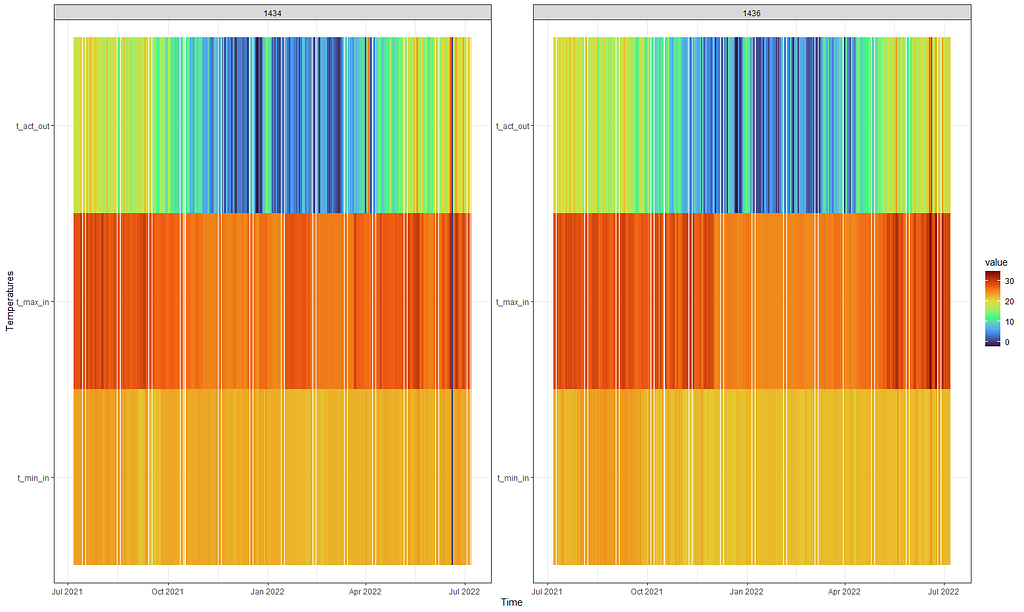Using the turbo colors from viridis, you can easily spot how the outside temperature shifts while inside it stays stable.

Perhaps the temperature itself is not THAT important but rather the difference between. So let’s plot that as well, taking a different coloring scheme from viridis.

day%>%
dplyr::filter(!lcn_id %in% c(‘1435’))%>%
dplyr::filter_at(vars(animals_start), all_vars(!is.na(.)))%>%
dplyr::select(lcn_id, date_interval, t_min_in, t_max_in)%>%
replace(is.na(.), 0)%>%
dplyr::mutate(diff=t_max_in-t_min_in)%>%
dplyr::select(lcn_id, date_interval, diff)%>%
ggplot(.,
aes(x=date_interval,
y=lcn_id,
fill=diff))+
geom_tile()+
scale_fill_viridis_c(option=”cividis”)+
labs(x=”Time”,
y=”Barn”,
fill=”Difference Min_Max”)+
theme_bw()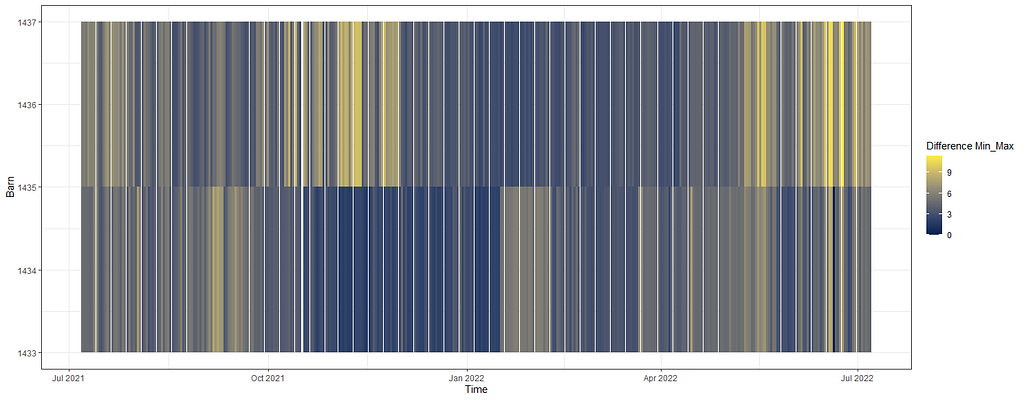Here you can see the differences between min and max per location. There definitely is a difference per day and that difference is also shifting. So the temperature is probably not at alle stable, but I am not sure if that makes any difference.

Below, I will show you a way to place everything into one chart — events and temperature combined.

day%>%
dplyr::filter(!lcn_id %in% c(‘1435’))%>%
dplyr::filter_at(vars(animals_start), all_vars(!is.na(.)))%>%
dplyr::select(lcn_id, date_interval, animals_dead, t_min_in, t_max_in, t_act_out)%>%
replace(is.na(.), 0)%>%
ggplot(.,
aes(x=date_interval))+
geom_line(aes(y=t_max_in), col=”red”)+
geom_line(aes(y=t_min_in), col=”blue”)+
geom_line(aes(y=t_act_out), col=”black”)+
col=”purple”,alpha=0.3, size=1)+
facet_wrap(~lcn_id, scales=”free”)+
labs(x=”Time”,
y=”Temperature in the barn”)+
theme_bw()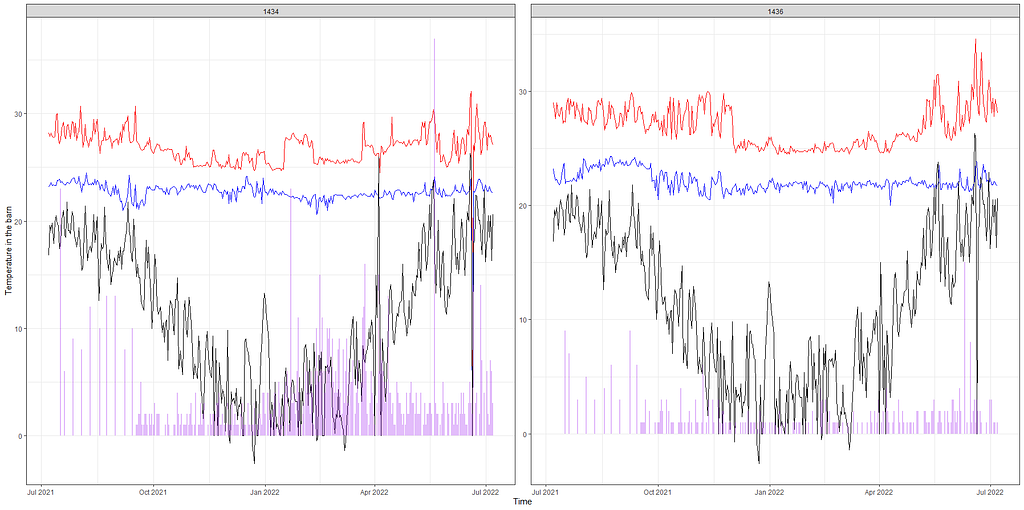The graph looks nice but it is impossible to detect any pattern from such a graph. It is not even clear if such a pattern exists.

Let’s combined the Poisson model predictions with the data and see if that will help us. Compared to the graph above the Poisson model should provide us with splines and I want to see if they follow the temperature. Do note that the model DOES NOT contain temperature of difference in temperature as a predictor. Not yet.

df_pois<-day%>%
dplyr::filter(!lcn_id %in% c(‘1435’))%>%
dplyr::filter_at(vars(animals_start), all_vars(!is.na(.)))%>%
dplyr::mutate(day = lubridate::day(date_interval),
month=lubridate::month(date_interval))%>%
t_min_in, t_max_in, t_act_out)%>%
replace(is.na(.), 0)
splines::ns(day,5)*as.factor(month)+as.factor(lcn_id),
data=df_pois)
pred_fit<-exp(predict(fit,newdata=df_pois))
df_fit_combined<-cbind(df_pois, pred_fit)
ggplot(df_fit_combined,
aes(x=day,
y=pred_fit,
group=lcn_id,
))+
geom_line(col=”orange”, size=1.5)+
geom_line(aes(y=t_max_in), col=”red”)+
geom_line(aes(y=t_min_in), col=”blue”)+
geom_line(aes(y=t_act_out), col=”black”)+
facet_grid(lcn_id~month)+
labs(x=”Day in month”,
y=”Predicted Counts of Mortality”,
col=”Predicted Deaths”)+
theme_bw()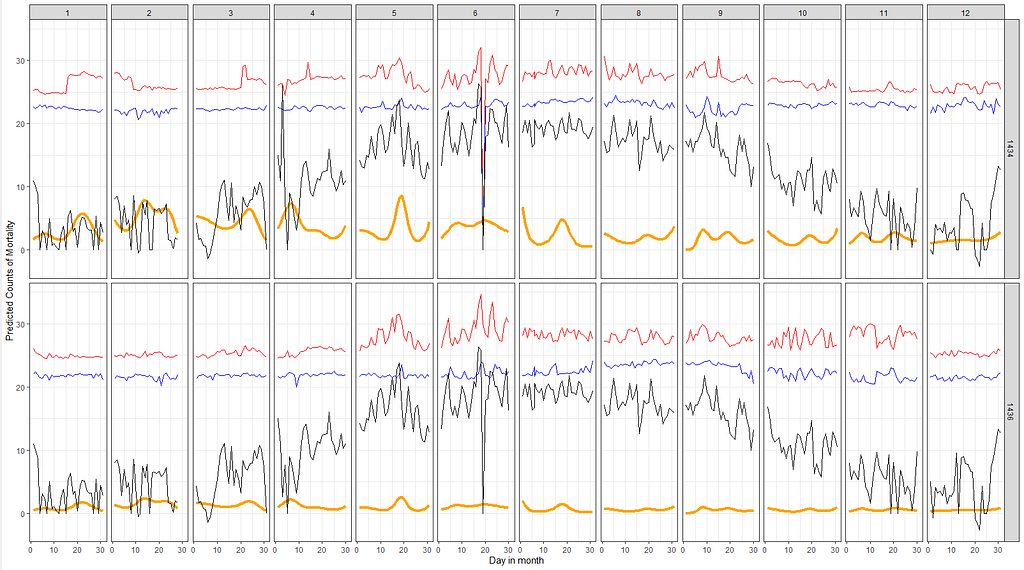Predictions are in orange and the red, blue and black lines show the maximum and minimum temp inside and the actual temp outside, respectively. Not clear to see any patterns. Sure, one location is predicted to have more events happening, but unclear how that relates to the actual temperature in- or outside of the barn.

Next up I want to see if I can connect the actual temperature data to the events that took place. I will add splines here as well which will make the model heavy but we have sufficient spread to get it done. At least, I hope.

df_pois<-day%>%
dplyr::filter(!lcn_id %in% c(‘1435’))%>%
dplyr::filter_at(vars(animals_start), all_vars(!is.na(.)))%>%
dplyr::mutate(day = lubridate::day(date_interval),
month=lubridate::month(date_interval))%>%
t_min_in, t_max_in, t_act_out)%>%
replace(is.na(.), 0)
splines::ns(day,5)*as.factor(month)+
splines::ns(t_min_in,5)+
splines::ns(t_max_in,5)+
as.factor(lcn_id),
data=df_pois)
summary(fit)
pred_fit<-exp(predict(fit,newdata=df_pois))
df_fit_combined<-cbind(df_pois, pred_fit)
ggplot(df_fit_combined,
aes(x=t_min_in,
y=t_max_in,
z=pred_fit))+
geom_contour_filled()+
labs(x=”Minimum Temp in Barn”,
y=”Maximum Temp in Barn”,
z=”Predicted deaths”)+
theme_bw()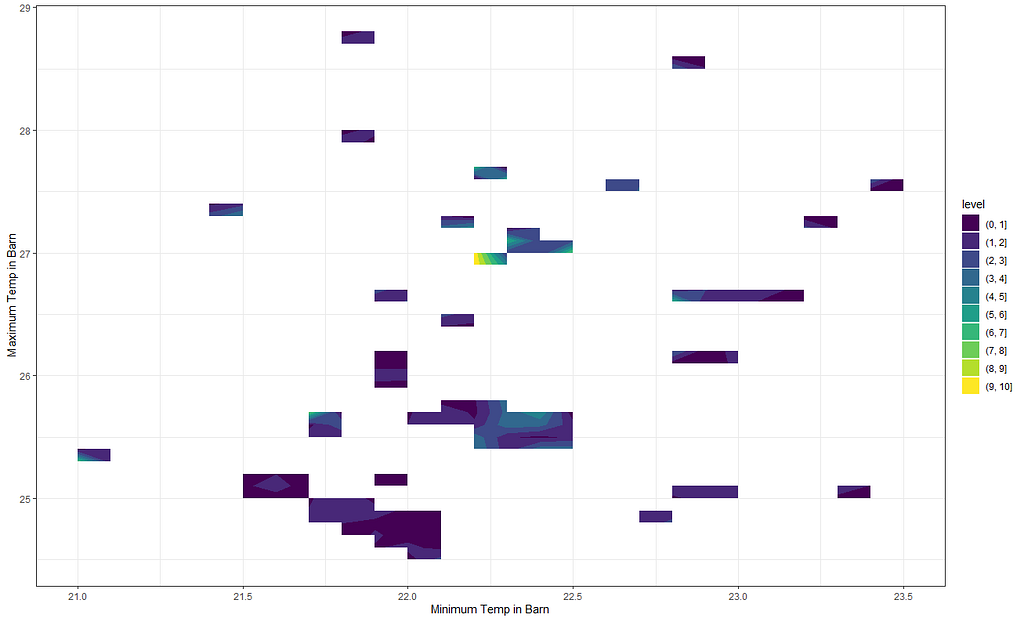Well, it seems there is not enough spread. What you are seeing is an attempt at a spline plot, or response surface if you will, in which I connect the minimum and maximum temperature to the number of events. Perhaps, the inclusion of day made it a bit infeasible. Let’s try again.

But first, I will plot the raw data to see why I have so many gaps.

ggplot(df_pois,
aes(x=t_min_in,
y=t_max_in))+
geom_point()+
labs(x=”Minimum Temp in Barn”,
y=”Maximum Temp in Barn”)+
theme_bw()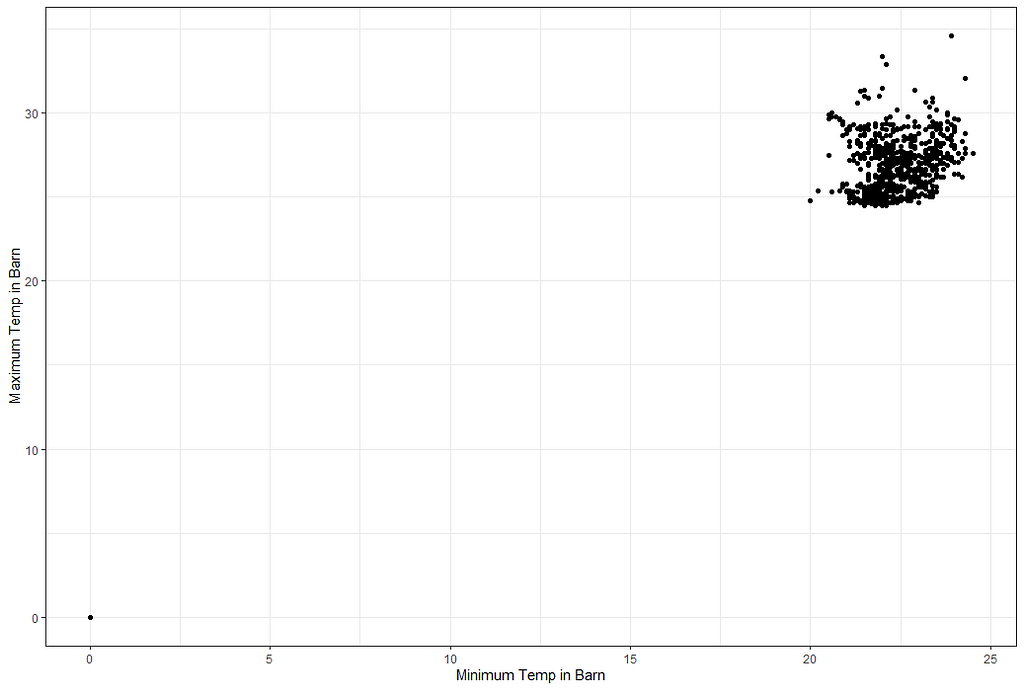There is sufficient spread, but the zero needs to go.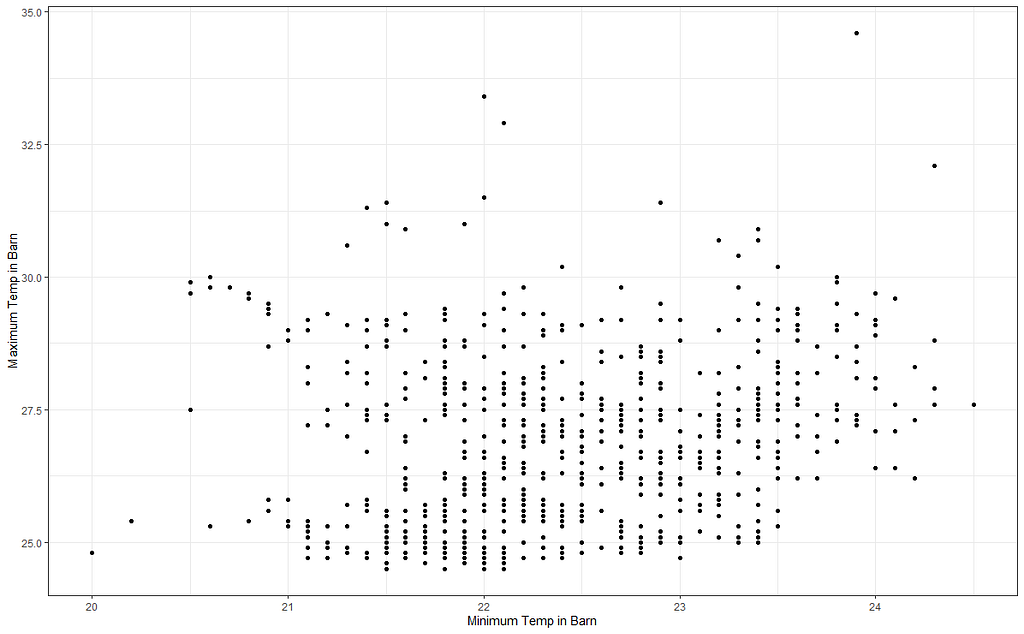Not a full screen, but, there should be more than what I am seeing now.

I will try again, deleting some data, to see if I can get a better view now. As you can see I am doing a lot of dplyr actions now. I just love dplyr!

df_pois<-day%>%
dplyr::filter(!lcn_id %in% c(‘1435’))%>%
dplyr::filter_at(vars(animals_start), all_vars(!is.na(.)))%>%
dplyr::mutate(day = lubridate::day(date_interval),
month=lubridate::month(date_interval))%>%
t_min_in, t_max_in, t_act_out)%>%
replace(is.na(.), 0)%>%
dplyr::filter(t_min_in>0)%>%
dplyr::filter(t_max_in>0)
splines::ns(t_max_in,5)+
as.factor(lcn_id),
data=df_pois)
summary(fit)
pred_fit<-exp(predict(fit,newdata=df_pois))
df_fit_combined<-cbind(df_pois, pred_fit)
ggplot(df_fit_combined,
aes(x=t_min_in,
y=t_max_in,
z=pred_fit))+
geom_contour_filled()+
geom_point(data=df_pois, alpha=0.1)+
labs(x=”Minimum Temp in Barn”,
y=”Maximum Temp in Barn”,
z=”Predicted deaths”)+
theme_bw()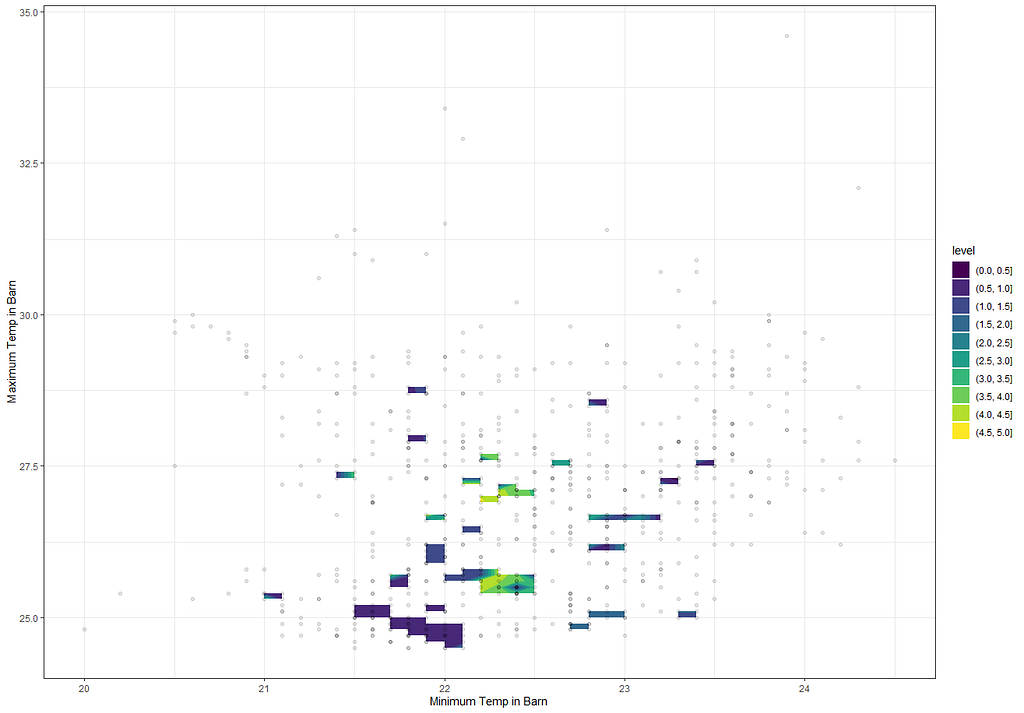Okay, so this is disappointing. Lets see if can get a better grip on understanding why the response surface will show more than it does not because IT DOES differentiate.

Perhaps I need to plot differently. The problem with contour plots is that it looks for connections and hence we will get gaps if no such connection is feasible. Let’s go for a different way of visualizing, using geom_raster.

ggplot(df_fit_combined,
aes(x=t_min_in,
y=t_max_in,
fill=pred_fit))+
geom_raster()+
scale_fill_viridis_c()+
labs(x=”Minimum Temp in Barn”,
y=”Maximum Temp in Barn”,
fill=”Predicted deaths”)+
theme_bw()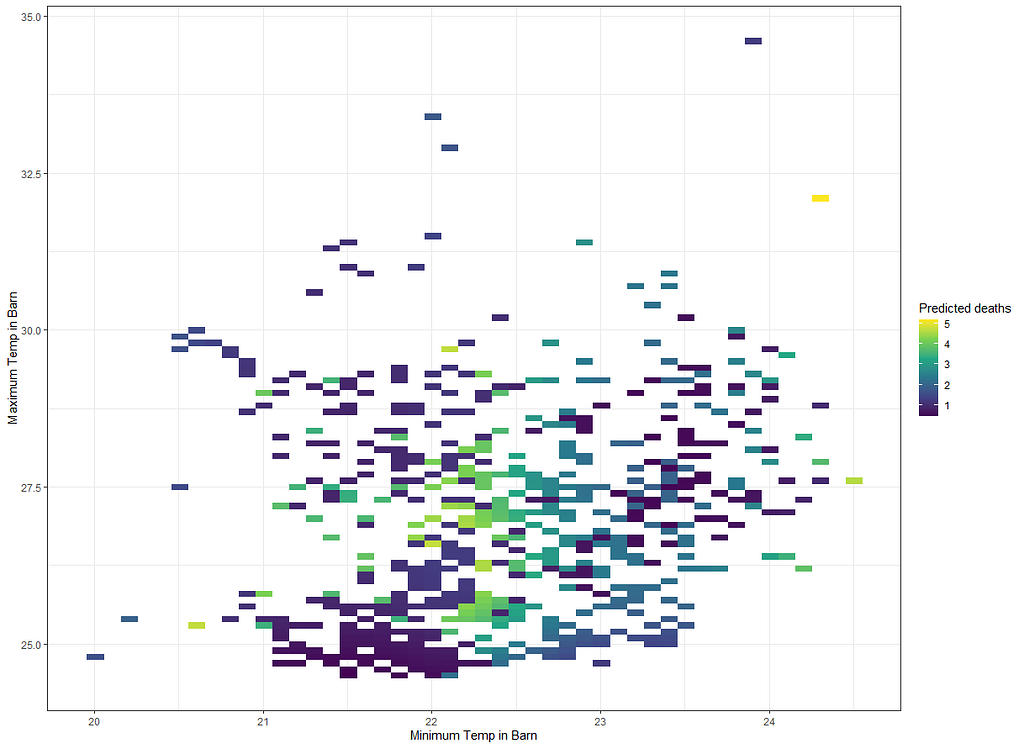Looking much better already. We can compare it to the original data as well.
g1<-ggplot(df_fit_combined,
aes(x=t_min_in,
y=t_max_in,
fill=pred_fit))+
geom_raster()+
scale_fill_viridis_c()+
labs(x=”Minimum Temp in Barn”,
y=”Maximum Temp in Barn”,
fill=”Predicted deaths”)+
theme_bw()+
theme(legend.position=”bottom”)
g2<-ggplot(df_fit_combined,
aes(x=t_min_in,
y=t_max_in,
geom_raster()+
scale_fill_viridis_c()+
labs(x=”Minimum Temp in Barn”,
y=”Maximum Temp in Barn”,
fill=”Predicted deaths”)+
theme_bw()+
theme(legend.position=”bottom”)
grid.arrange(g1,g2,ncol=2)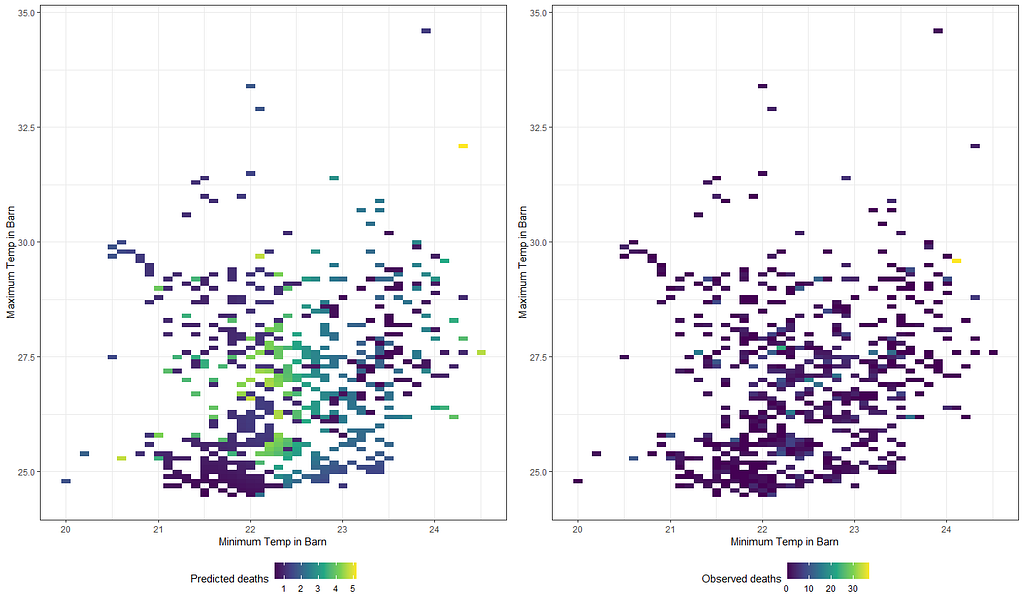Can not say this model is doing much of a good job. In the original data, there seems to be more extreme events happening than what I predict. Now, extreme events are a pain to model in general, but this is more of difference than what I feel comfortable with.

Will it stick if I differentiate per location?

g3<-ggplot(df_fit_combined,
aes(x=t_min_in,
y=t_max_in,
fill=pred_fit))+
geom_raster()+
scale_fill_viridis_c()+
labs(x=”Minimum Temp in Barn”,
y=”Maximum Temp in Barn”,
fill=”Predicted deaths”)+
theme_bw()+
facet_wrap(~lcn_id, scales=”free”)+
theme(legend.position=”right”)
g4<-ggplot(df_fit_combined,
aes(x=t_min_in,
y=t_max_in,
geom_raster()+
scale_fill_viridis_c()+
labs(x=”Minimum Temp in Barn”,
y=”Maximum Temp in Barn”,
fill=”Observed deaths”)+
theme_bw()+
facet_wrap(~lcn_id, scales=”free”)+
theme(legend.position=”right”)
grid.arrange(g3,g4,ncol=1)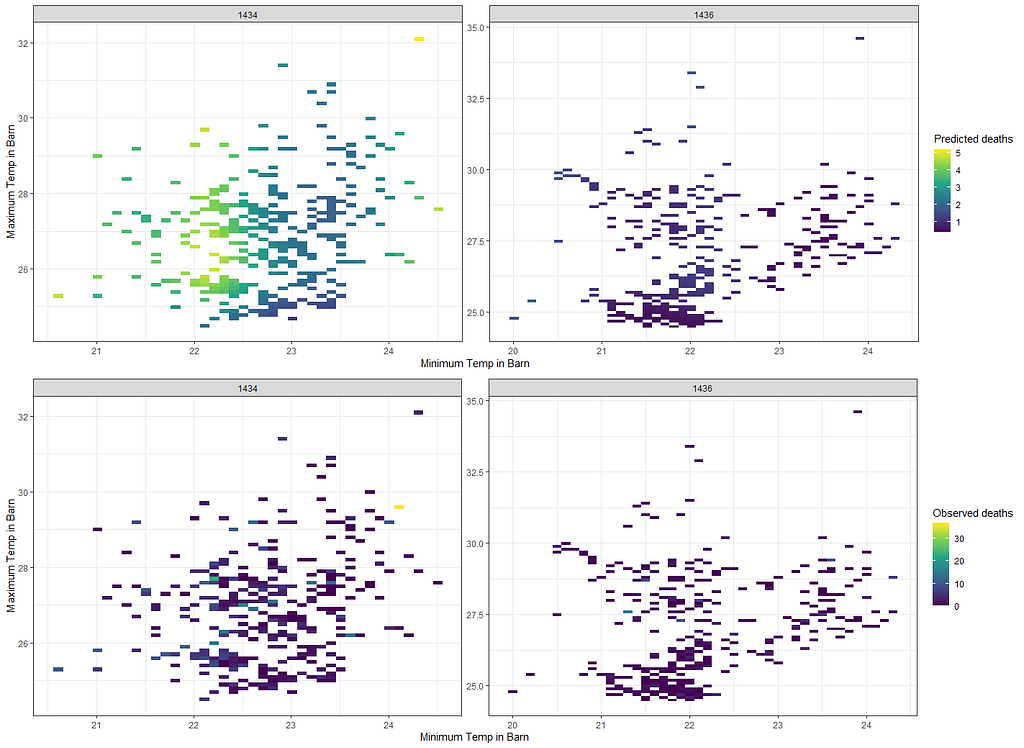At least I can see where the issue is.

Alright, so let’s park this and move to the difference between temperatures. Perhaps this will give us more ease in modeling.

df_pois<-day%>%
dplyr::filter(!lcn_id %in% c(‘1435’))%>%
dplyr::filter_at(vars(animals_start), all_vars(!is.na(.)))%>%
replace(is.na(.), 0)%>%
dplyr::mutate(diff=t_max_in-t_min_in,
day = lubridate::day(date_interval),
month=lubridate::month(date_interval))%>%
dplyr::select(lcn_id, day, month, diff, animals_dead, date_interval)
splines::ns(day,5)*
splines::ns(diff,5)+
as.factor(month)+
as.factor(lcn_id),
data=df_pois)
summary(fit)
pred_fit<-exp(predict(fit,newdata=df_pois))
df_fit_combined<-cbind(df_pois, pred_fit)
ggplot(df_fit_combined,
aes(x=date_interval,
y=diff,
size=pred_fit,
col=pred_fit))+
geom_point(alpha=0.7)+
scale_color_viridis_c(option=”magma”)+
facet_wrap(~lcn_id)+
labs(x=”Date”,
y=”Difference Temp in Barn”,
size=”Predicted deaths”)+
theme_bw()+
theme(legend.position=”bottom”)+
guides(color = FALSE)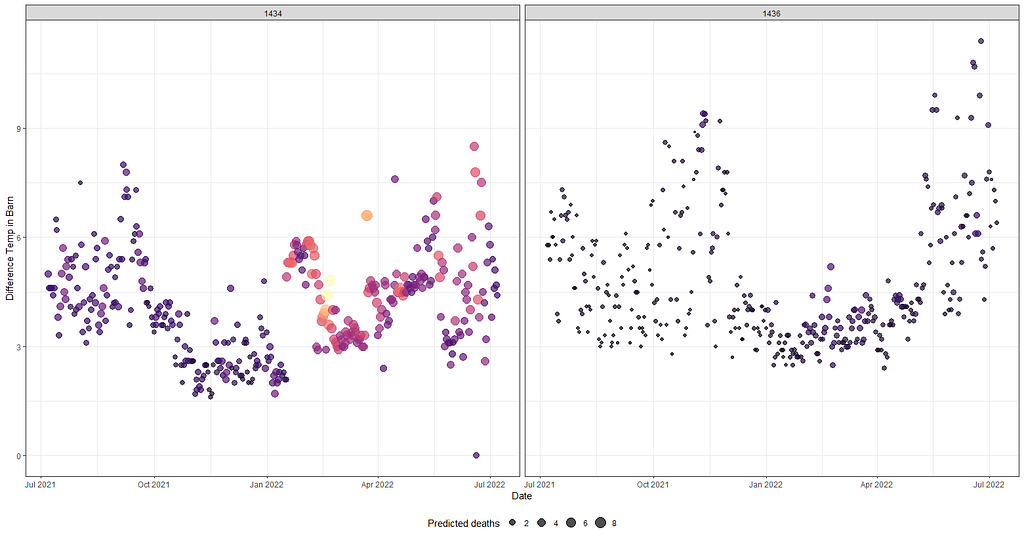So what I am showing here is, over time en per location, the predicted deaths a function of the temperature difference. The size and color both indicate the number of predicted deaths. Always good to compare this plot to the observed data.
ggplot(df_fit_combined,
aes(x=date_interval,
y=diff,
geom_point(alpha=0.7)+
scale_color_viridis_c(option=”magma”)+
facet_wrap(~lcn_id)+
labs(x=”Date”,
y=”Difference Temp in Barn”,
size=”Observed deaths”)+
theme_bw()+
theme(legend.position=”bottom”)+
guides(color = FALSE)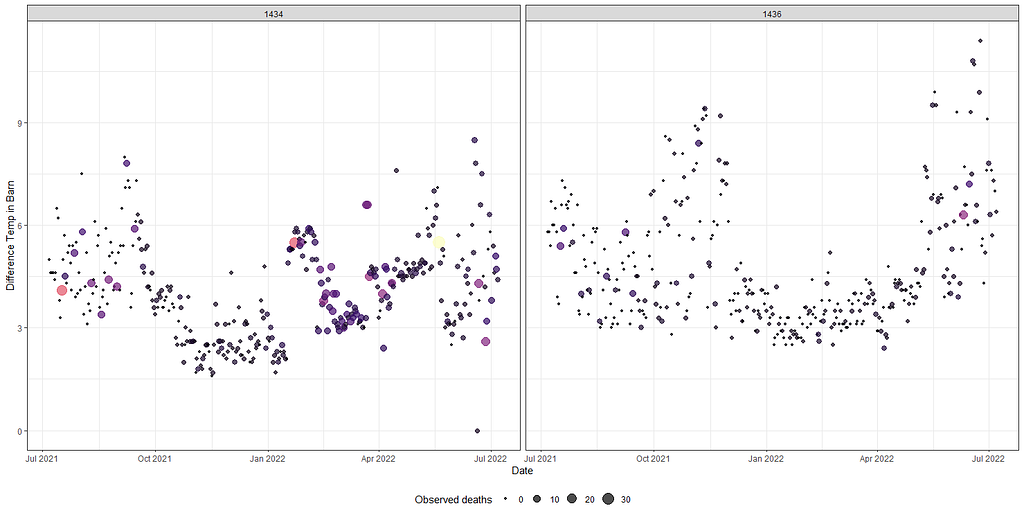Once again, the observed values look different then the predicted values. It seems the model did not go along with the extremes.

A decent way to look at the performance of a model is to draw up a calibration plot.

ggplot(df_fit_combined,
y=pred_fit))+
geom_point(alpha=0.7)+
geom_abline(intercept = 0, slope=1, lty=2, col=”red”)+
facet_grid(lcn_id~month, scales=”free”)+
labs(x=”Observed deaths”,
y=”Predicted deaths”)+
theme_bw()+
theme(legend.position=”bottom”)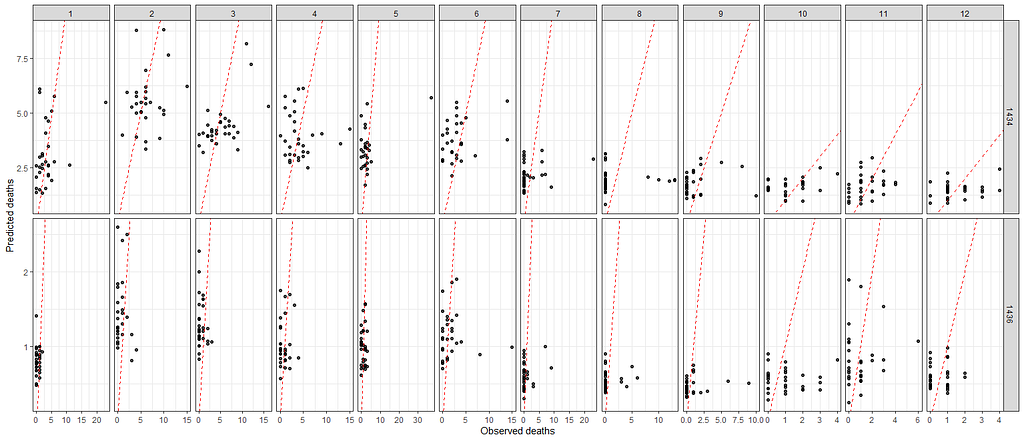It does not seem to bad at first, but the fact that the calibration line is not moving diagonal already hints at scale ranges that are too far. Lets create a more clear picture.
ggplot(df_fit_combined,
y=pred_fit))+
geom_point(alpha=0.3)+
geom_abline(intercept = 0, slope=1, lty=2, col=”red”)+
geom_smooth(method=’lm’, lty=2, col=”blue”, se=FALSE)+
facet_grid(lcn_id~month, scales=”free”)+
labs(x=”Observed deaths”,
y=”Predicted deaths”)+
theme_bw()+
theme(panel.grid.major = element_blank(), panel.grid.minor = element_blank(),
panel.background = element_blank(), axis.line = element_line(colour = “black”))+
theme(legend.position=”bottom”)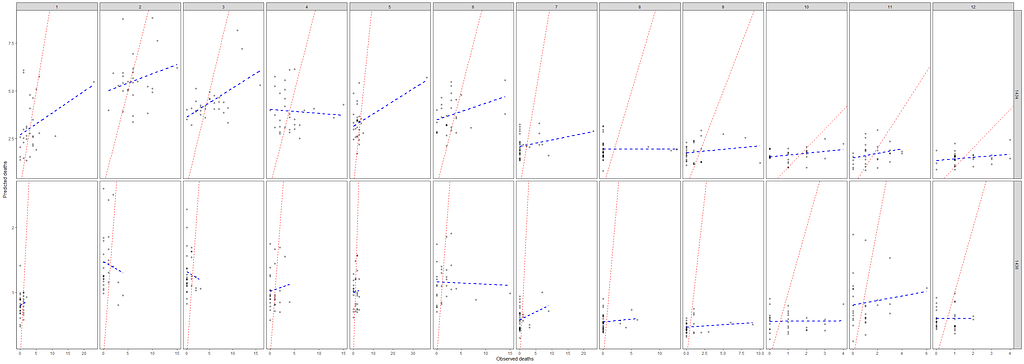Does not look good at all! In fact, these predictions are MESSED UP. No wonder the rest did not look really good.

Okay, so we need to improve and I will start first by getting rid of the Poisson distribution. It was a nice start, but time to explore others like the Negative Binomial. For that, I will use the MASS package which has glm.nb.

df_pois<-day%>%
dplyr::filter(!lcn_id %in% c(‘1435’))%>%
dplyr::filter_at(vars(animals_start), all_vars(!is.na(.)))%>%
replace(is.na(.), 0)%>%
dplyr::mutate(diff=t_max_in-t_min_in,
day = lubridate::day(date_interval),
month=lubridate::month(date_interval))%>%
dplyr::select(lcn_id, day, month, diff, animals_dead, date_interval)
splines::ns(day,5)*
splines::ns(diff,5)+
as.factor(month)+
as.factor(lcn_id),
data=df_pois)
summary(fit)
pred_fit<-exp(predict(fit,newdata=df_pois))
df_fit_combined<-cbind(df_pois, pred_fit)
ggplot(df_fit_combined,
y=pred_fit))+
geom_point(alpha=0.3)+
geom_abline(intercept = 0, slope=1, lty=2, col=”red”)+
geom_smooth(method=’lm’, lty=2, col=”blue”, se=FALSE)+
facet_grid(lcn_id~month, scales=”free”)+
labs(x=”Observed deaths”,
y=”Predicted deaths”)+
theme_bw()+
theme(panel.grid.major = element_blank(), panel.grid.minor = element_blank(),
panel.background = element_blank(), axis.line = element_line(colour = “black”))+
theme(legend.position=”bottom”)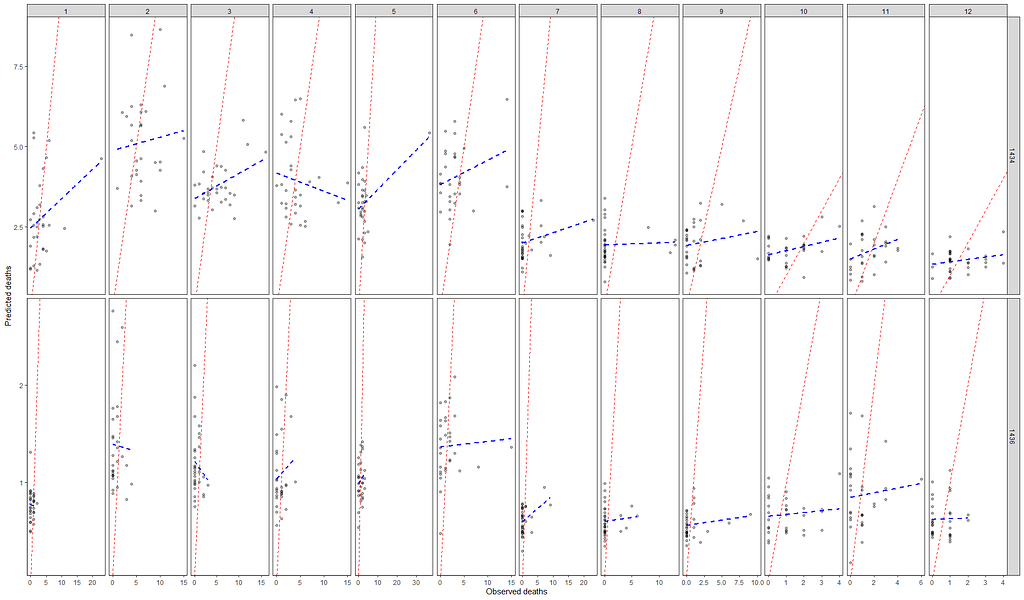Can’t say that I am too excited. For some reason, I am not able to capture the data.

Perhaps, I should deal with the zero inflation which I am suspecting I stepped over because of the focus on date, day, and time.

fill=as.factor(lcn_id)))+
geom_histogram(alpha=0.5, bins=20)+
theme_bw()+
y=”Count”,
fill=”Location”)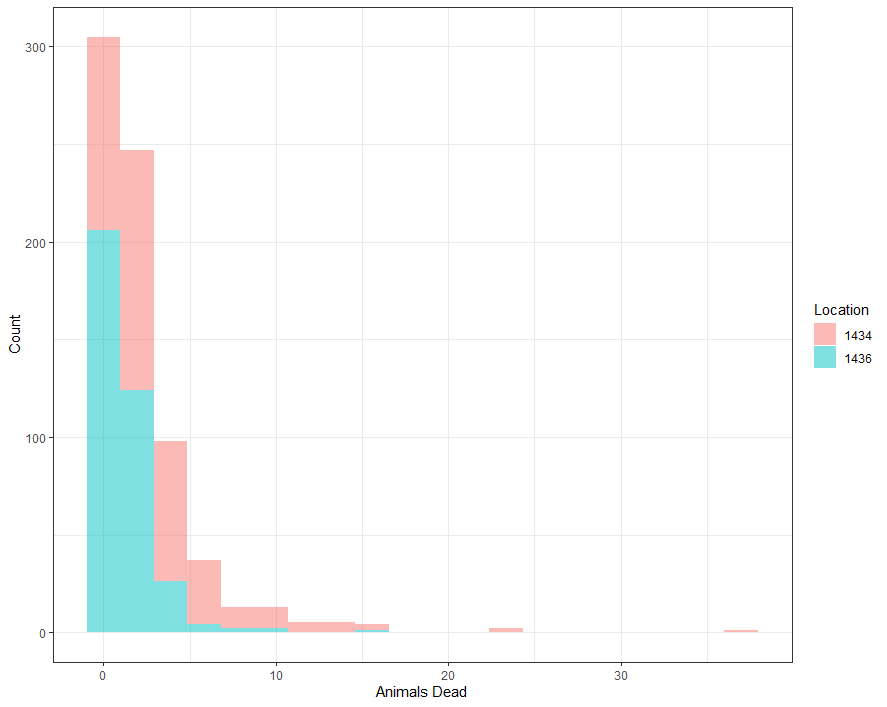Oooops! I should have done that first, a bit stupid of me actually. Now, it all falls to place. THIS is severe zero-inflation and needs to be dealt with.

I have recently posted about zero-inflated models, analyzing them in a Bayesian way. They are not easy as they break up the model into two — one part to predict a zero or non-zero death and the other on how many non-zero’s. I will use the pscl package for a standard glm approach to a zero-inflated Poisson model. What you will see me do is copy the previous model, but also add a specific model for the zero part. Here, I chose month.

splines::ns(day,5)*
splines::ns(diff,5)+
as.factor(month)+
as.factor(lcn_id) | month, data = df_pois)
summary(fit)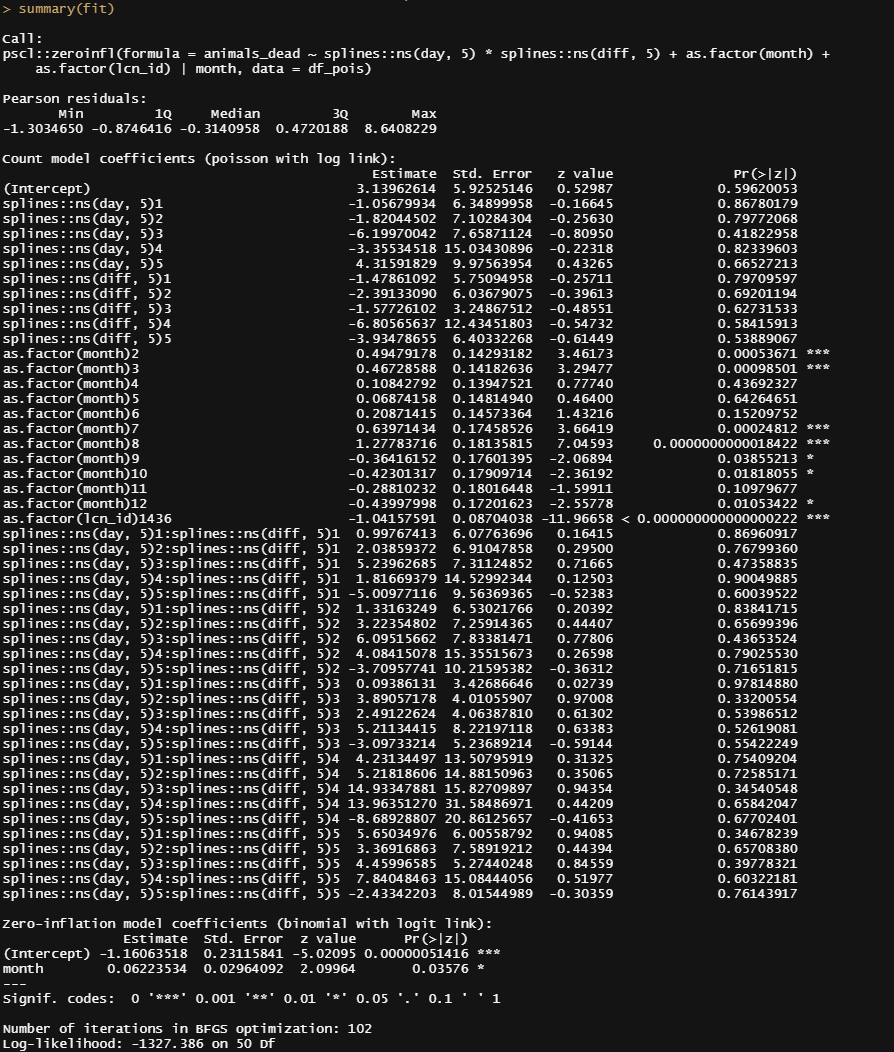And now, let’s check performance.

pred_fit<-predict(fit,newdata=df_pois)
df_fit_combined<-cbind(df_pois, pred_fit)
ggplot(df_fit_combined,
y=pred_fit))+
geom_point(alpha=0.3)+
geom_abline(intercept = 0, slope=1, lty=2, col=”red”)+
geom_smooth(method=’lm’, lty=2, col=”blue”, se=FALSE)+
facet_grid(lcn_id~month, scales=”free”)+
labs(x=”Observed deaths”,
y=”Predicted deaths”)+
theme_bw()+
theme(panel.grid.major = element_blank(), panel.grid.minor = element_blank(),
panel.background = element_blank(), axis.line = element_line(colour = “black”))+
theme(legend.position=”bottom”)
ggplot(df_fit_combined)+
geom_histogram(aes(x=pred_fit), fill=”blue”, alpha=0.5)+
facet_grid(lcn_id~month, scales=”free”)+
labs(x=”Day”,
y=”Deaths”,
main=”Predicted (blue) vs Observed (red) Deaths from a Zero-Inflated Poisson model”)+
theme_bw()

The model runs but checking the predicted vs the observed deaths does not really give me the feeling this model is doing any better. Perhaps it is time to recheck the model in its entirety because that location parameter, lcn_id, seems to have a massive main impact but in this model, I have nothing to interact it with.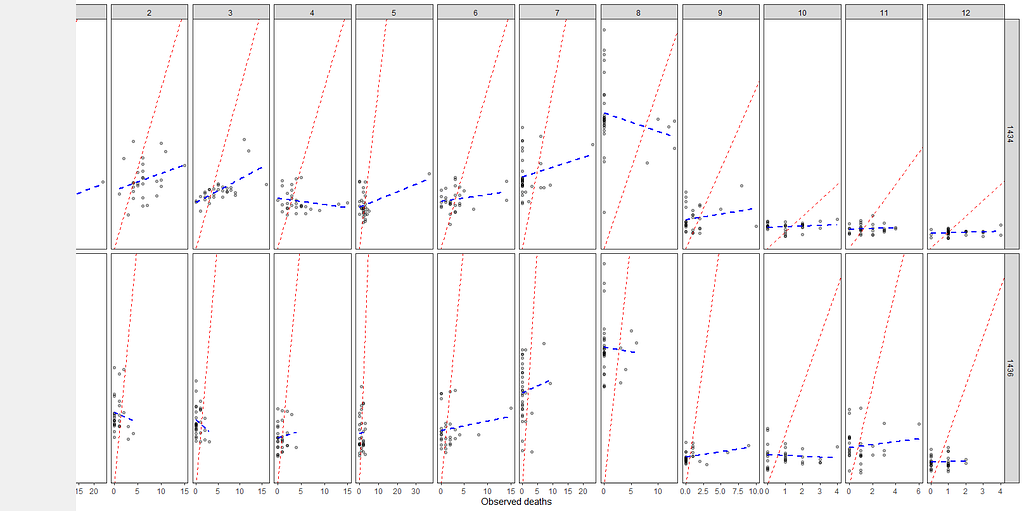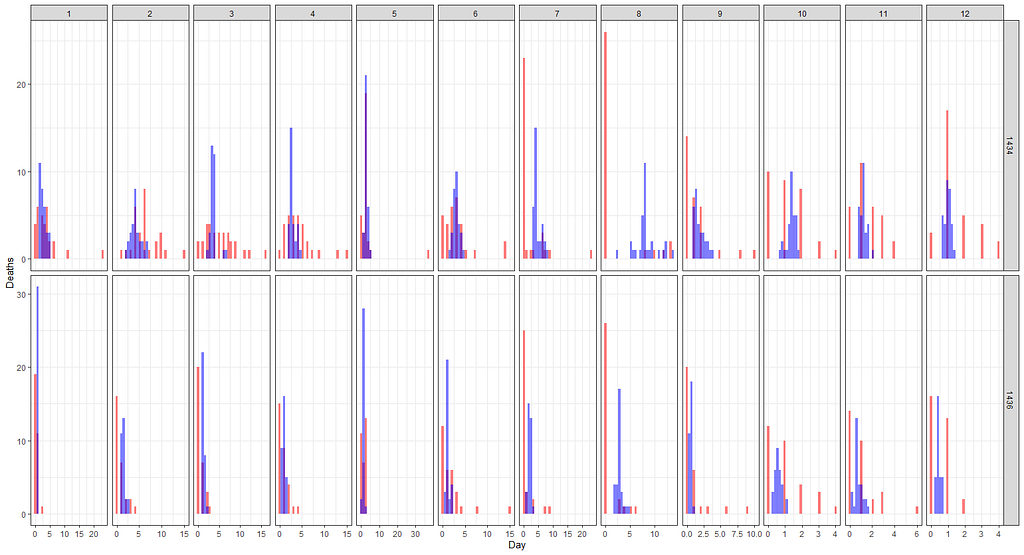splines::ns(day,5)*
splines::ns(diff,5)+
as.factor(month) +
splines::ns(day,5):as.factor(month) +
as.factor(lcn_id) | month, data = df_pois)
summary(fit2)
AIC(fit); AIC(fit2)
pred_fit<-predict(fit2,newdata=df_pois)
df_fit_combined<-cbind(df_pois, pred_fit)
ggplot(df_fit_combined,
y=pred_fit))+
geom_point(alpha=0.3)+
geom_abline(intercept = 0, slope=1, lty=2, col=”red”)+
geom_smooth(method=’lm’, lty=2, col=”blue”, se=FALSE)+
labs(x=”Observed deaths”,
y=”Predicted deaths”)+
theme_bw()+
theme(panel.grid.major = element_blank(), panel.grid.minor = element_blank(),
panel.background = element_blank(), axis.line = element_line(colour = “black”))+
theme(legend.position=”bottom”)+
ylim(0,50)
ggplot(df_fit_combined)+
geom_histogram(aes(x=pred_fit), fill=”blue”, alpha=0.5)+
labs(x=”Deaths”,
y=”Frequency”,
title=”Predicted (blue) vs Observed (red) Deaths from a Zero-Inflated Poisson model”)+
theme_bw()+
xlim(0,30)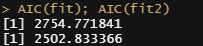The model interacting day with month is a MUCH better fit according to the AIC.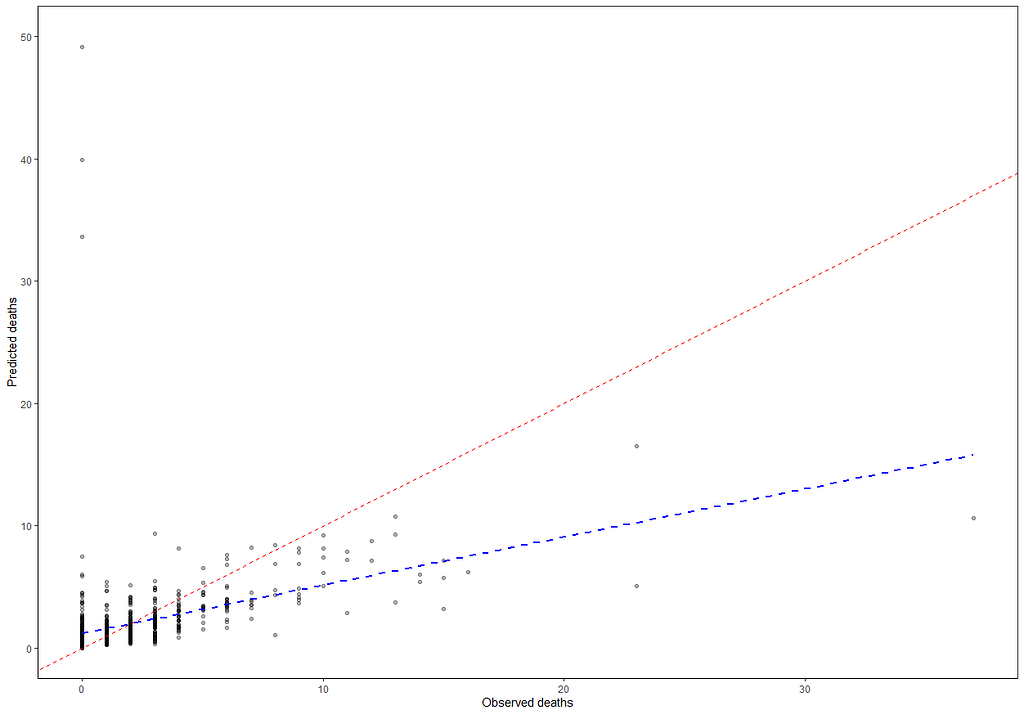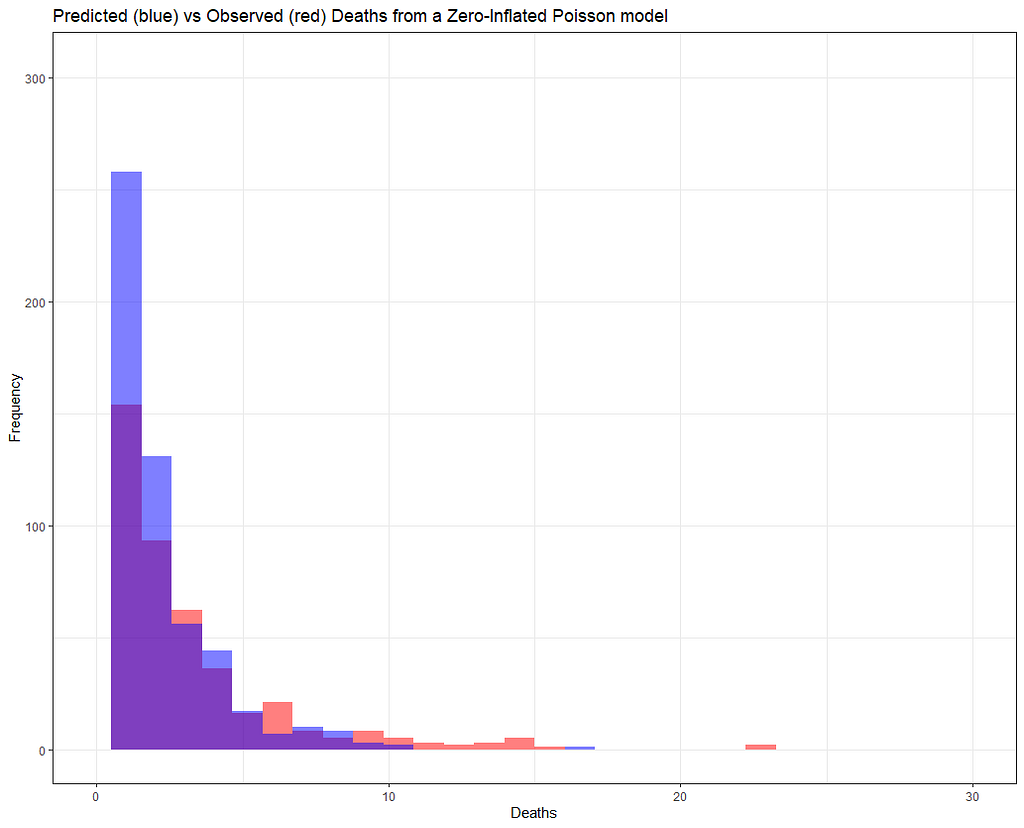But still, enough room for improvement as well. It is really not that easy to predict event data such as this.

ggplot(df_fit_combined)+
geom_histogram(aes(x=pred_fit), fill=”blue”, alpha=0.5)+
facet_grid(lcn_id~month, scales=”free”)+
labs(x=”Day”,
y=”Frequency”,
main=”Predicted (blue) vs Observed (red) Deaths from a Zero-Inflated Poisson model”)+
theme_bw()+
xlim(0,30)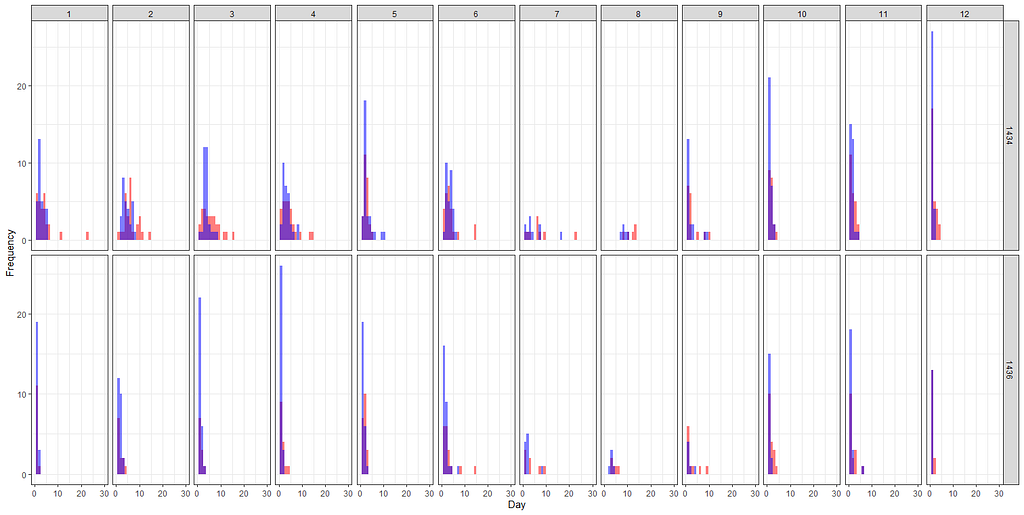Let’s add day as a variable to the zero part.

splines::ns(day,5)*
splines::ns(diff,5)+
as.factor(month) +
splines::ns(day,5):as.factor(month) +
as.factor(lcn_id) | day + month,
dist=”poisson”,
data = df_pois)
summary(fit3)
AIC(fit); AIC(fit2); AIC(fit3)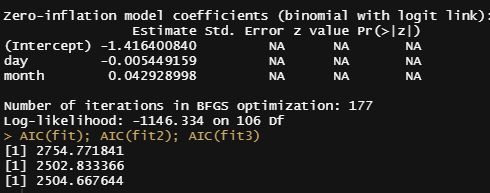That did not go well.

And we will do another round of modeling, this time referring back to the original temperatures and including more interactions, now with the location. Because we know that day, month and location differentiate the data.

df_pois<-day%>%
dplyr::filter(!lcn_id %in% c(‘1435’))%>%
dplyr::filter_at(vars(animals_start), all_vars(!is.na(.)))%>%
dplyr::mutate(day = lubridate::day(date_interval),
month=lubridate::month(date_interval))%>%
t_min_in, t_max_in, t_act_out)%>%
replace(is.na(.), 0)%>%
dplyr::filter(t_min_in>0)%>%
dplyr::filter(t_max_in>0)
splines::ns(day,5)*
splines::ns(t_min_in,5)+
splines::ns(t_max_in,5)+
splines::ns(t_act_out,5)+
as.factor(month) +
splines::ns(day,5):as.factor(month)+
as.factor(lcn_id) + as.factor(month):as.factor(lcn_id)|
day + month,
dist=”poisson”,
data = df_pois)
summary(fit4)
AIC(fit); AIC(fit2); AIC(fit3); AIC(fit4)
pred_fit<-predict(fit4,newdata=df_pois)
df_fit_combined<-cbind(df_pois, pred_fit)
ggplot(df_fit_combined,
y=pred_fit))+
geom_point(alpha=0.3)+
geom_abline(intercept = 0, slope=1, lty=2, col=”red”)+
geom_smooth(method=’lm’, lty=2, col=”blue”, se=FALSE)+
labs(x=”Observed deaths”,
y=”Predicted deaths”)+
theme_bw()+
theme(panel.grid.major = element_blank(), panel.grid.minor = element_blank(),
panel.background = element_blank(), axis.line = element_line(colour = “black”))+
theme(legend.position=”bottom”)+
ylim(0,30)+
xlim(0,30)
ggplot(df_fit_combined,
y=pred_fit))+
geom_point(alpha=0.3)+
geom_abline(intercept = 0, slope=1, lty=2, col=”red”)+
geom_smooth(method=’lm’, lty=2, col=”blue”, se=FALSE)+
labs(x=”Observed deaths”,
y=”Predicted deaths”)+
facet_grid(lcn_id~month, scales=”free”)+
theme_bw()+
theme(panel.grid.major = element_blank(), panel.grid.minor = element_blank(),
panel.background = element_blank(), axis.line = element_line(colour = “black”))+
theme(legend.position=”bottom”)+
ylim(0,10)+
xlim(0,10)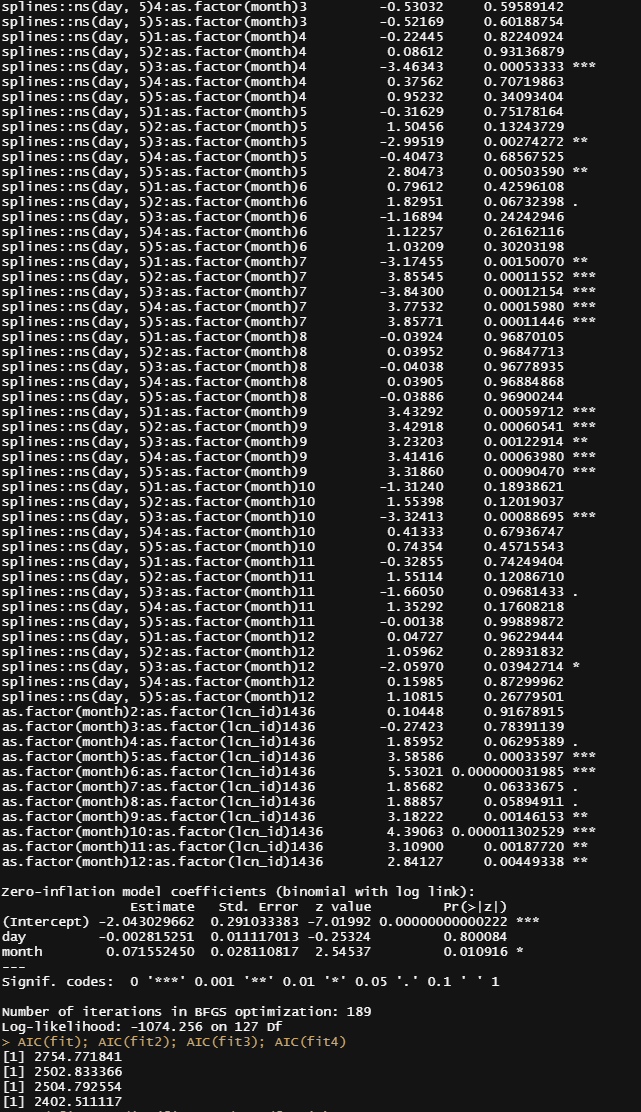BIG model, but the AIC is lowest.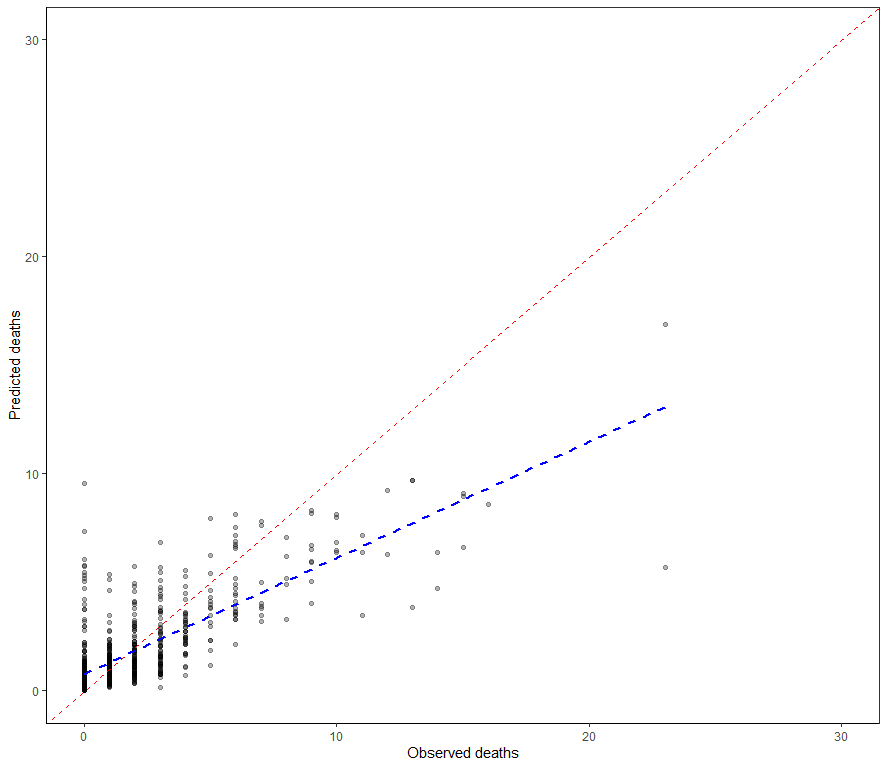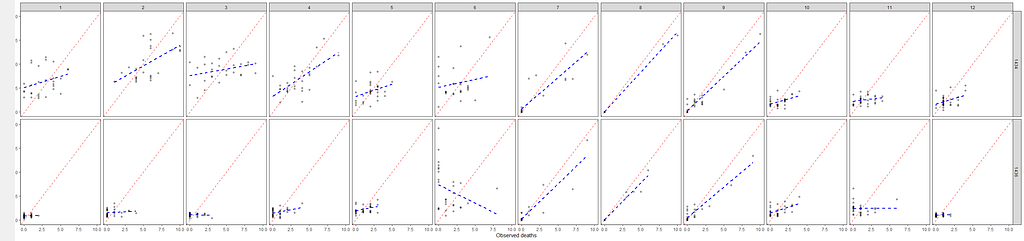Predictions still not really better.

It is safe to say that I will get anywhere close to where I want to go if I keep up this way. Diverting to the negative-binomial really did not do anything to shift the predictive power of the model. So, what I want to do is now is see if I can get more signal from the temperature data which means applying decomposition methods.

If we look back at the temperature data it is easy to see there is much going on, but what I tried to do up until now is cover that with a 5-knot splines. Lets just see how that looks. Remember, a 5-knot natural spline means 7 degrees of freedom.

day%>%
dplyr::filter(!lcn_id %in% c(‘1435’))%>%
dplyr::filter_at(vars(animals_start), all_vars(!is.na(.)))%>%
t_min_in, t_max_in, t_act_out)%>%
replace(is.na(.), 0)%>%
dplyr::filter(t_min_in>0)%>%
dplyr::filter(t_max_in>0)%>%
ggplot(., aes(x=date_interval,
y=t_max_in))+
geom_line()+
geom_smooth(method = “lm”,
formula = y ~ splines::ns(x, df = 7))+
facet_wrap(~lcn_id)+
theme_bw()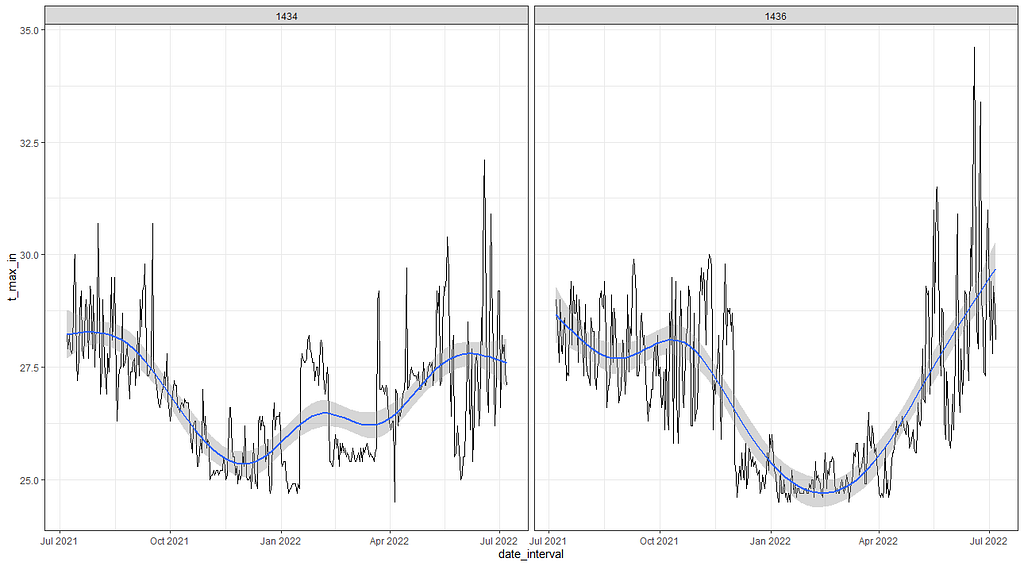Immediately shows that temporal data should not be approximated with splines. There is so much more that can be done.

So, let’s see if we can use time-series decomposition. I will start with a univariate time series, taking the minimum temperature at barn 1436. Then I will run a decomp on it.

df<-day%>%
dplyr::filter(lcn_id==’1436′)%>%
dplyr::filter_at(vars(animals_start), all_vars(!is.na(.)))%>%
replace(is.na(.), 0)
min(lubridate::date(df\$date_interval))
max(lubridate::date(df\$date_interval))
myts<-ts(df\$t_min_in,
start=c(lubridate::year(min(df\$date_interval)),lubridate::yday(min(df\$date_interval))),
end=c(lubridate::year(max(df\$date_interval)),lubridate::yday(max(df\$date_interval))),
frequency=365)
class(myts)
plot(myts)
decomp<-stats::decompose(myts)
plot(decomp)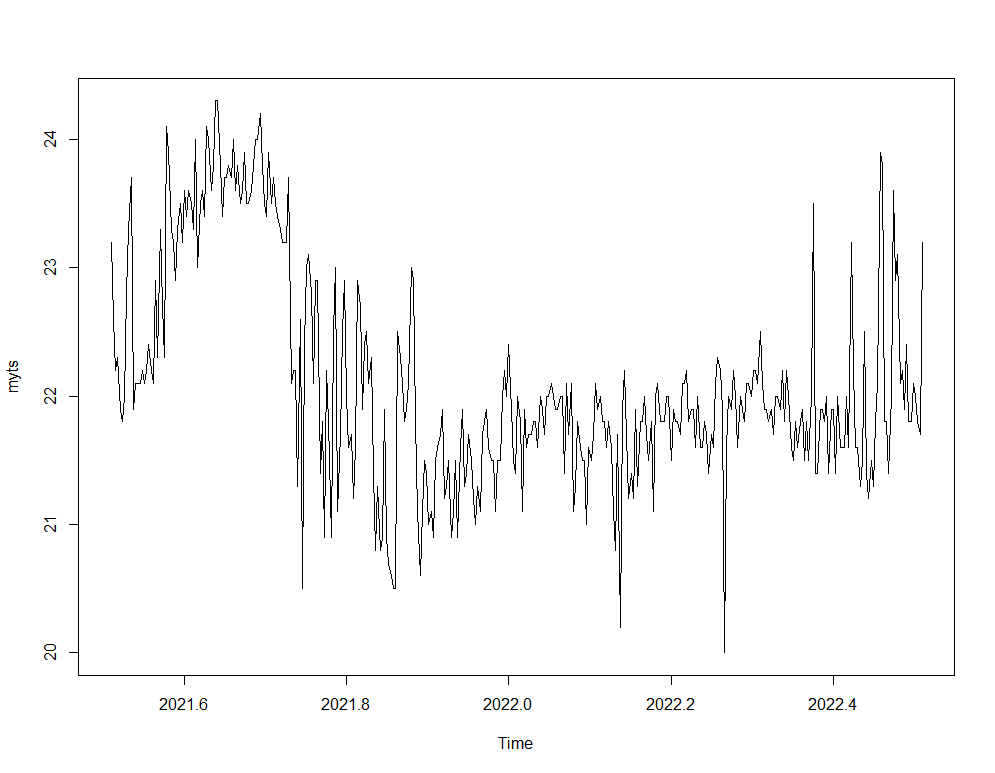The ts obect of the minimum temperature at barn 1436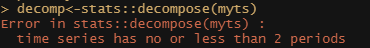Unfortunately, a decomp is not possible since we are missing sufficient periods. Not sure WHY this is necessary, because we a full year worth of data, but my guess is that it has to do with the seasonal component. Which we cannot calculate since we only have a single year.

I found another method to look for extracting components, especially cycles, called the Hodrick-Prescott filter. First time I heard about it, so let’s see what it does. Its application is pretty easy as are most R functions. Like I said before, there is surely a danger in that.

p_min_temp<-mFilter::hpfilter(myts, freq = 1600)
plot(hp_min_temp)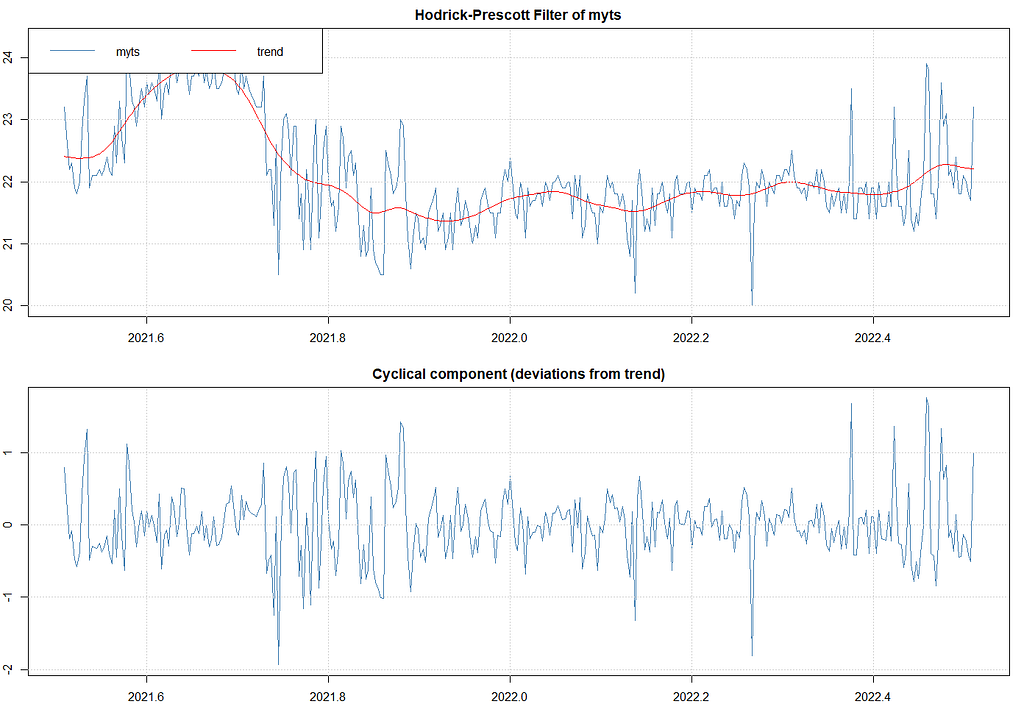The data, the trend and the cyclical component which are deviations from the trend.

The above looks good, but I remembered that there is an even better method which is to analyze the univariate time series via Unobserved Component Models (UCMs). I have blogged about that method before and what I like about it is that it works exactly like the decomposition method, with the exception that you can tweak the components, set them to zero or fixed variances, and this model with them. Let’s give that a shot.

Below, I will run a UCM where I ask for level, slope, and cycle components. The cycle period is set at 12, which means 12 days. I am also asking for an irregular component that mimics irregularities which are basically deviations.

ucm_min_temp<-rucm::ucm(t_min_in~0,
data=df,
level=TRUE,
slope=TRUE,
cycle=TRUE,cycle.period = 12,
irregular=TRUE)
ucm_min_temp
g1<-ggplot(df,
aes(x=date_interval))+
geom_line(aes(y=t_min_in))+
geom_line(aes(y=ucm_min_temp\$s.level), col=”red”, size=1)+
labs(x=”Date”,
y=”Min Temp in the Barn”,
title=”UCM-Level”)+
theme_bw()+
theme(axis.text.y=element_blank())
g2<-ggplot(df,
aes(x=date_interval))+
geom_line(aes(y=ucm_min_temp\$s.cycle), col=”blue”, size=0.5)+
labs(x=”Date”,
y=”Min Temp in the Barn”,
title=”UCM-cycle”)+
theme_bw()+
theme(axis.text.y=element_blank())
g3<-ggplot(df,
aes(x=date_interval))+
geom_line(aes(y=ucm_min_temp\$s.slope), col=”seagreen”, size=0.5)+
labs(x=”Date”,
y=”Min Temp in the Barn”,
title=”UCM-Slope”)+
theme_bw()+
theme(axis.text.y=element_blank())
grid.arrange(g1,g2,g3, nrow=3)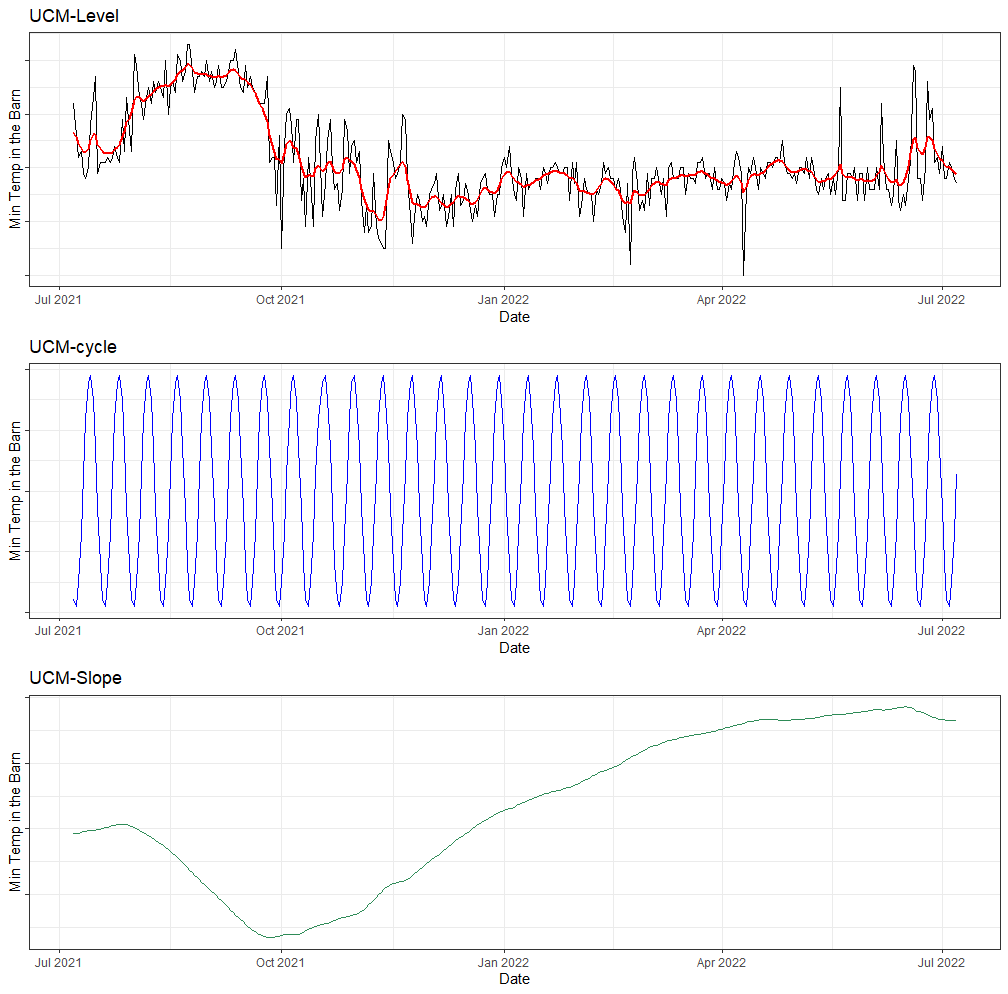The level, cycle and slope. The cycle could for sure use some tweaking and perhaps I should have set it at 7, meaning 7 days, or 30 meaning a month. However, we a talking temperature here, so any kind of cycle is more seasonal or daily. Since we only have the average per day, the season is all we have.

Lets plot the level component of the minimum temperature with the actual mortality data and see if we can find any pattern.

df\$level<-ucm_min_temp\$s.level
data=df, type=”l”)
obj2<-lattice::xyplot(level~date_interval,
data=df, type=”l”)
latticeExtra::doubleYScale(obj1,
obj2,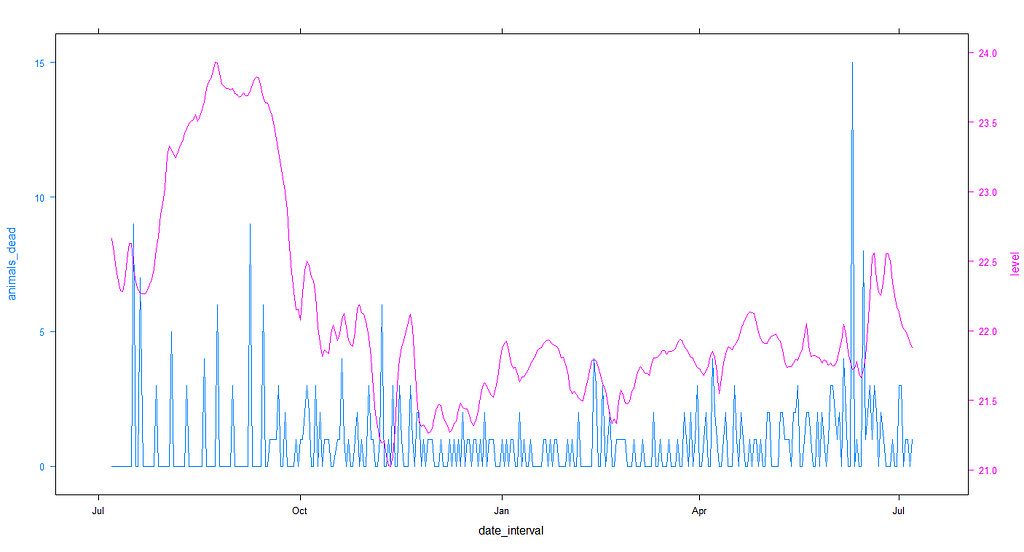Picture showing the minimum temperature and number of deaths per data for location 1436. Difficult to find any pattern by naked eye.
df<-day%>%
dplyr::filter(lcn_id==’1434′)%>%
dplyr::filter_at(vars(animals_start), all_vars(!is.na(.)))%>%
replace(is.na(.), 0)
min(lubridate::date(df\$date_interval))
max(lubridate::date(df\$date_interval))
ucm_min_temp<-rucm::ucm(t_min_in~0,
data=df,
level=TRUE,
slope=TRUE,
cycle=TRUE,cycle.period = 12,
irregular=TRUE)
ucm_min_temp
df\$level<-ucm_min_temp\$s.level
data=df, type=”l”)
obj2<-lattice::xyplot(level~date_interval,
data=df, type=”l”)
latticeExtra::doubleYScale(obj1,
obj2,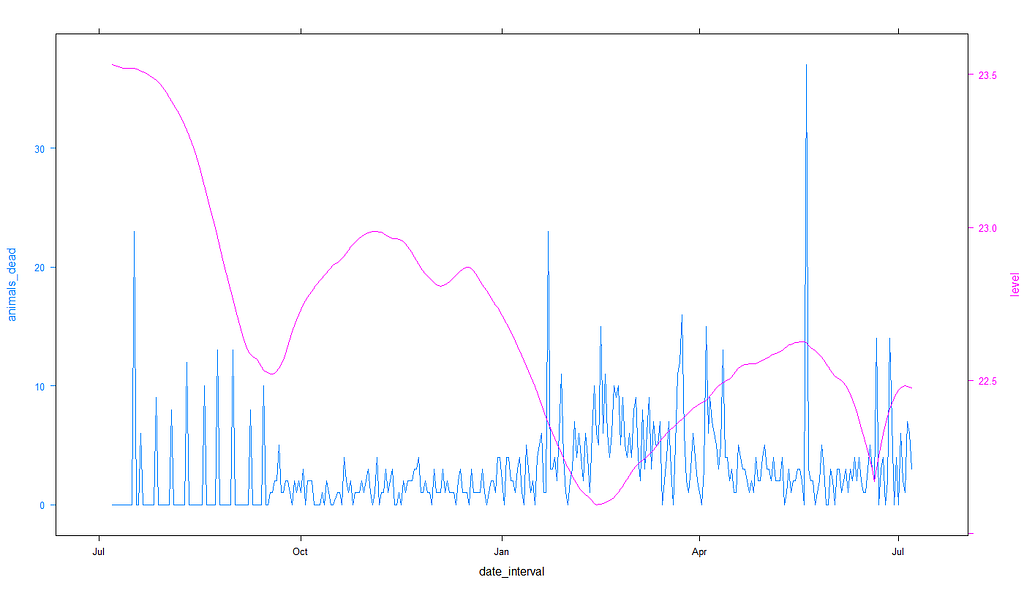Picture showing the minimum temperature and number of deaths per data for location 1434. Difficult to find any pattern by naked eye.

So, what now? We can see that the pattern of mortality shifts per location, and that the for sure is mortality to be seen. There are events, no matter what. A next step could be to revert back to original survival analysis, like Kaplan-Meier or Cox Regression, since both can incorporate time-varying coefficients like time. We could then add parametric survival analysis to look for the expected mortality curve.

First, let’s good back to the last model we made, and draw the predicted survival curve to get another glimpse of the performance of the model. Just to get a better glimpse of what we have.

df_pois<-day%>%
dplyr::filter(!lcn_id %in% c(‘1435’))%>%
dplyr::filter_at(vars(animals_start), all_vars(!is.na(.)))%>%
dplyr::mutate(day = lubridate::day(date_interval),
month=lubridate::month(date_interval))%>%
dplyr::select(lcn_id, date_interval, day, month, animals_dead, animals_start,
t_min_in, t_max_in, t_act_out)%>%
replace(is.na(.), 0)%>%
dplyr::filter(t_min_in>0)%>%
dplyr::filter(t_max_in>0)
splines::ns(day,5)*
splines::ns(t_min_in,5)+
splines::ns(t_max_in,5)+
splines::ns(t_act_out,5)+
as.factor(month) +
splines::ns(day,5):as.factor(month)+
as.factor(lcn_id) + as.factor(month):as.factor(lcn_id)|
day + month,
dist=”negbin”,
data = df_pois)
summary(fit5)
pred_fit<-predict(fit5,newdata=df_pois)
df_fit_combined<-cbind(df_pois, pred_fit)
plot(hist(df_fit_combined\$pred_fit))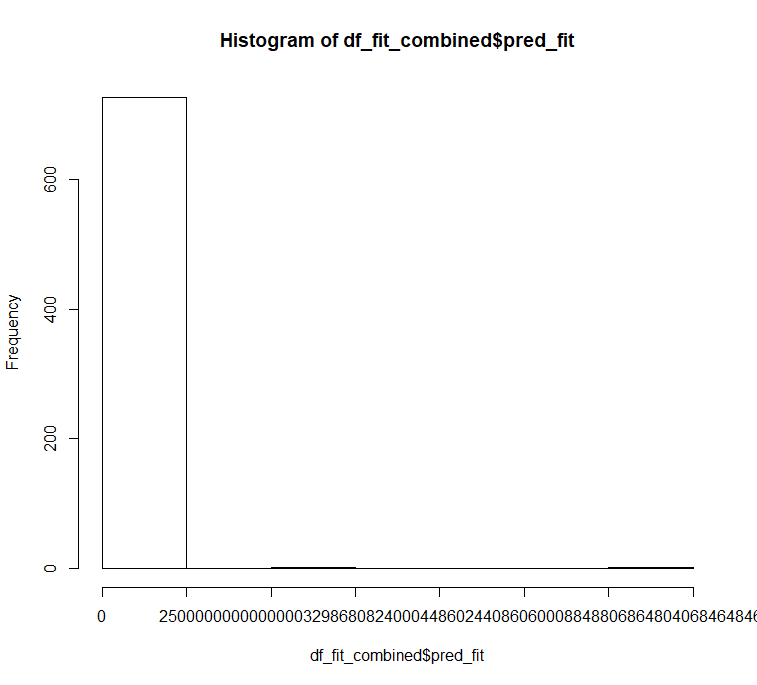Wow, there are SOME funky predictions over here!
ggplot(df_fit_combined,
aes(x=pred_fit, fill=as.factor(lcn_id)))+
geom_density(alpha=0.5)+
theme_bw()+
labs(fill=”Location”)+
xlim(0,40)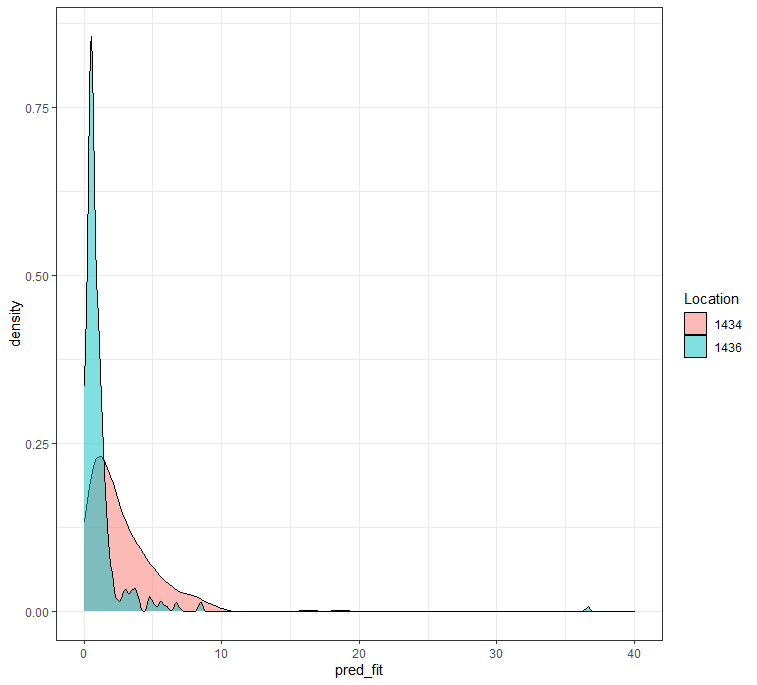If I set the cap at 40, it looks better. There is no specific reason for me to set the cap at 40, except that I know that sometimes the mortality at a single day can reach that number. Luckily, the model does do zero-inflation in its prediction and the predicted numbers are not that funky.

Remember, I warned all of you that this kind of modeling would NOT be easy. I purposefully refused to model the data using Kaplan-Meier or Cox Regression but instead chose to model the event data using time, and including the zero-inflation.

A better way to check how my model is doing is to take a look at the cumulative sum of the deaths I predicted and create a sort of liferisk plot. Remember, the real insane predictions I kick out and replace with zero here. I need to otherwise the plot will not work, but these are in fact predictions I discard. That IS a limitation to be sure.

df_fit_combined%>%
dplyr::group_by(lcn_id)%>%
dplyr::mutate(pred_fit = ifelse(pred_fit>40, NA, pred_fit))%>%
replace(is.na(.), 0)%>%
ggplot(., aes(x=date_interval))+
facet_wrap(~lcn_id, scales=”free”)+
theme_bw()
df_fit_combined%>%
dplyr::group_by(lcn_id)%>%
dplyr::mutate(pred_fit = ifelse(pred_fit>40, NA, pred_fit))%>%
replace(is.na(.), 0)%>%
ggplot(., aes(x=date_interval))+
facet_wrap(~lcn_id, scales=”free”)+
theme_bw()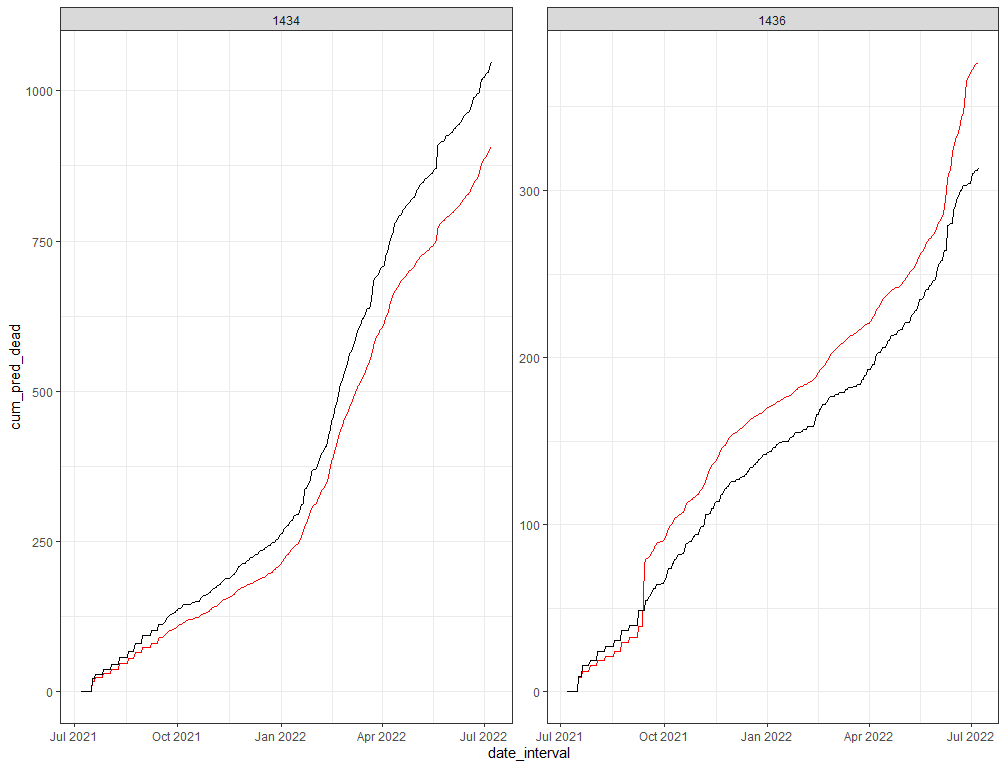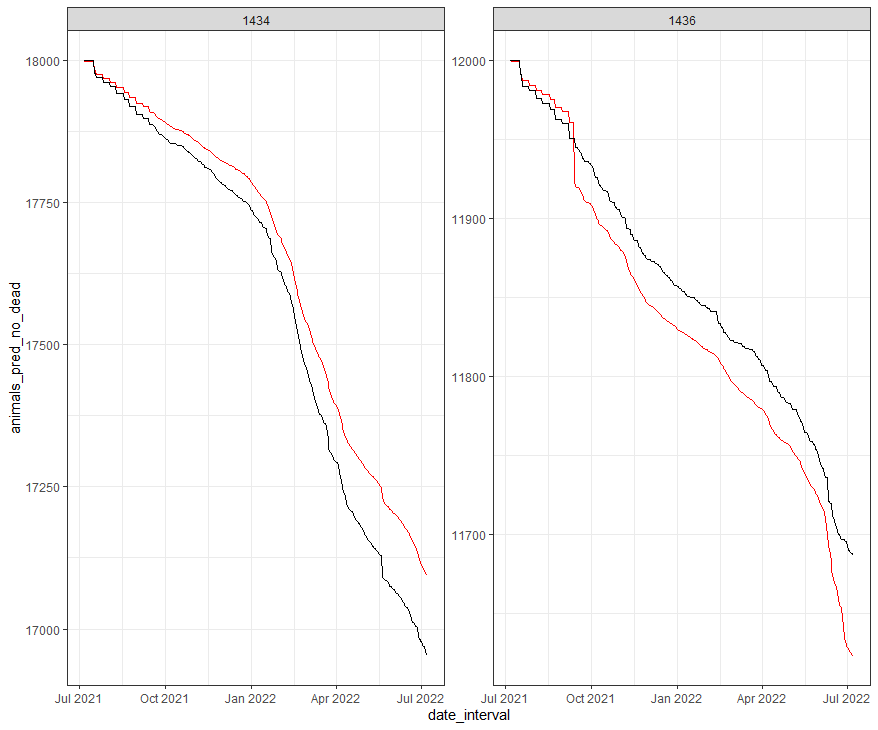Left: cumulative sum of deaths. Right: liferisk plot showing the decrease in animals. In both plots the predictions are in red and the actual numbers in black. You can see how the model over and under predicts, but since I did not use any variation you can not see the uncertainty. Lets add that as well.

The pscl package doe not have a standard confidence interval calculation, so the easiest way to get confidence intervals is to bootstrap the data. Also here, I need to delete severe predictions, which I do not like but let’s do it anyhow.

splines::ns(day,5)*
splines::ns(t_min_in,5)+
splines::ns(t_max_in,5)+
splines::ns(t_act_out,5)+
as.factor(month) +
splines::ns(day,5):as.factor(month)+
as.factor(lcn_id) + as.factor(month):as.factor(lcn_id)|
day + month,
dist=”negbin”,
data = df_pois,
X=TRUE,
y=TRUE,
model=TRUE,
stat <- function(df_pois, inds)
day + month)
predict(
pscl::zeroinfl(model,
dist = “negbin”,
data = df_pois[inds, ]))

(res <- boot::boot(df_pois, stat, R = 2000, parallel=”snow”, ncpus = 6))
CI <- setNames(as.data.frame(t(sapply(1:nrow(df_pois), function(row)
boot.ci(res, conf = 0.95, type = “basic”, index = row)\$basic[, 4:5]))),
c(“lower”, “upper”))
df_all <- cbind.data.frame(df_pois,
response = predict(fit5), CI)
df_boot<-df_all%>%
dplyr::mutate(response = ifelse(response>40, NA, response),
lower = ifelse(between(lower,-40,40), lower, NA),
upper = ifelse(between(upper,-40,40), upper, NA))
table(is.na(df_boot\$lower))
table(is.na(df_boot\$upper))
table(is.na(df_boot\$response))
ggplot(df_boot,
aes(x=as.numeric(response),
y=lubridate::date(date_interval)))+
geom_pointrange(aes(xmin=0, xmax=upper), alpha=0.5)+
geom_point()+
facet_wrap(~lcn_id, scales=”free”)+
theme_bw()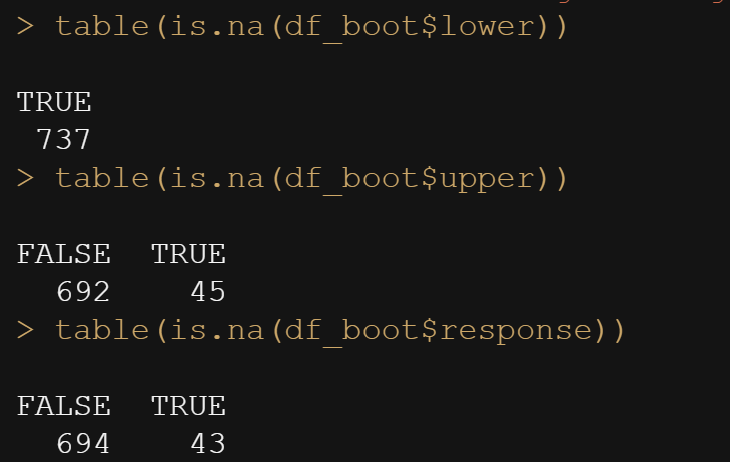As you can see, the lower bound on the confidence interval is really really low, so I will have to set them at zero. Normally, you do not want to have to do this and rather have the model respect the boundary of zero, but that did not happen here. So I helped out a bit.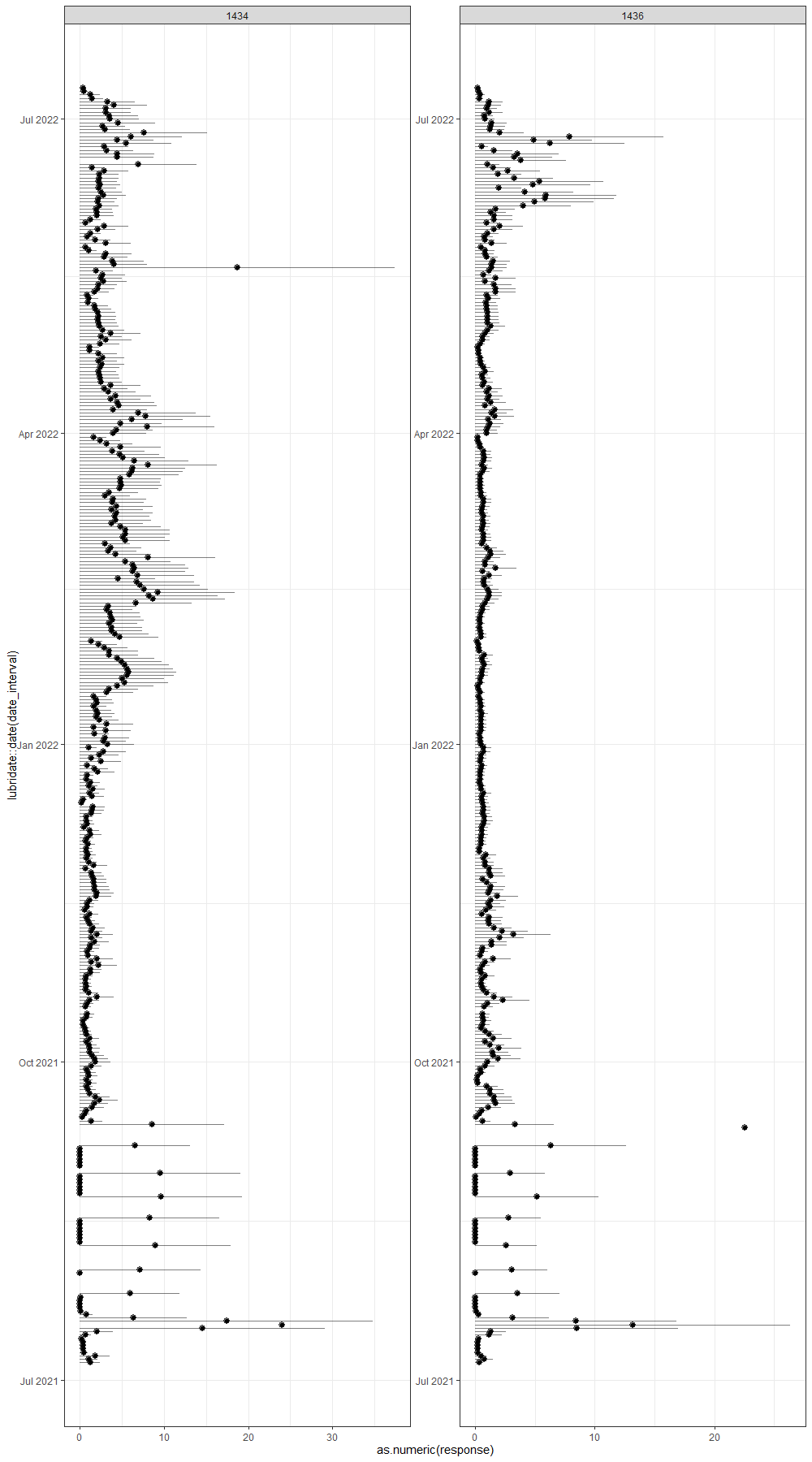And the bootstrapped predictions. Look not that bad, after helping out. You can also see that at certain points no prediction was made. Lets see how all of this looks in the cumulative sum plots.

And here below is the cumulative sum plot. Boy oh boy. Remember, I ran the model 2000 times and predicted, 2000 times, the number of deaths for each of the data points I observed. Then, I obtained the confidence intervals of each of those predictions. One could say these are prediction intervals. Then I started summing these up and what I get below is quite an honest accounting of how certain my model is about itself, and it ain’t very certain. Then again, I was not very certain to start with.

df_boot%>%
dplyr::group_by(lcn_id)%>%
dplyr::select(lcn_id, date_interval,
animals_start,
response,
lower,
upper)%>%
replace(is.na(.), 0)%>%
ggplot(., aes(x=date_interval))+
fill=”red”, alpha=0.3)+
facet_wrap(~lcn_id, scales=”free”)+
theme_bw()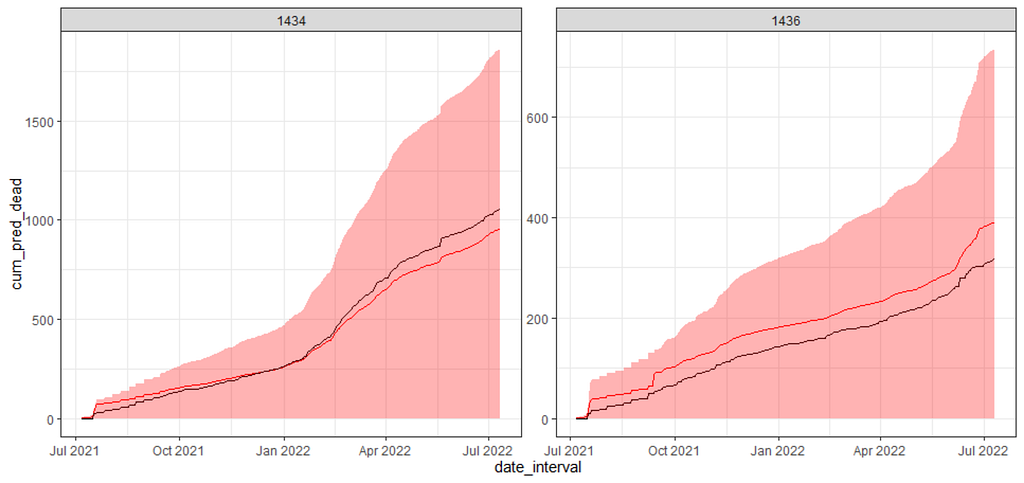The confidence intervals look good, in terms of how they should look, but they are huge. Of course, if yu would run a model the model would have to update itself, but still one can expect that it is difficult to get any kind of valid predictions going on when you try to model discrete data like this.

Now, there is one last thing I want to try and that is to take the curve from the UCM model, help me predict the events and then plot it. I will do that for one location only. Just to see. I do not expect much from it, but who knows. It is not as if I did not warn you guys from the beginning.

df_pois<-day%>%
dplyr::filter(!lcn_id %in% c(‘1435’))%>%
dplyr::filter_at(vars(animals_start), all_vars(!is.na(.)))%>%
dplyr::mutate(day = lubridate::day(date_interval),
month=lubridate::month(date_interval))%>%
dplyr::select(lcn_id, date_interval, day, month, animals_dead, animals_start,
t_min_in, t_max_in, t_act_out)%>%
replace(is.na(.), 0)%>%
dplyr::filter(t_min_in>0)%>%
dplyr::filter(t_max_in>0)
ucm_min_temp<-rucm::ucm(t_min_in~0,
data=df_pois,
level=TRUE,
slope=TRUE,
cycle=TRUE,cycle.period = 12,
irregular=TRUE)
df_pois\$level<-ucm_min_temp\$s.level
splines::ns(day,5)+
splines::ns(level,5)+
as.factor(month) +
as.factor(lcn_id)+
splines::ns(day,5):as.factor(month)+
splines::ns(day,5):splines::ns(level,5)+
as.factor(lcn_id) + as.factor(month):as.factor(lcn_id)|
day + month,
dist=”negbin”,
data = df_pois,
X=TRUE,
y=TRUE,
model=TRUE,
AIC(fit5);AIC(fit6)
stat <- function(df_pois, inds)
day + month)
predict(
pscl::zeroinfl(model,
dist = “negbin”,
data = df_pois[inds, ]))

(res <- boot::boot(df_pois, stat, R = 2000, parallel=”snow”, ncpus = 6))
CI <- setNames(as.data.frame(t(sapply(1:nrow(df_pois), function(row)
boot.ci(res, conf = 0.95, type = “basic”, index = row)\$basic[, 4:5]))),
c(“lower”, “upper”))
df_all <- cbind.data.frame(df_pois,
response = predict(fit6), CI)
df_boot<-df_all%>%
dplyr::mutate(response = ifelse(response>40, NA, response),
lower = ifelse(between(lower,-40,40), lower, NA),
upper = ifelse(between(upper,-40,40), upper, NA))
ggplot(df_boot,
aes(x=as.numeric(response),
y=lubridate::date(date_interval)))+
geom_pointrange(aes(xmin=0, xmax=upper), alpha=0.5)+
geom_point()+
facet_wrap(~lcn_id, scales=”free”)+
theme_bw()
df_boot%>%
dplyr::group_by(lcn_id)%>%
dplyr::select(lcn_id, date_interval,
animals_start,
response,
lower,
upper)%>%
replace(is.na(.), 0)%>%
ggplot(., aes(x=date_interval))+
fill=”red”, alpha=0.3)+
facet_wrap(~lcn_id, scales=”free”)+
theme_bw()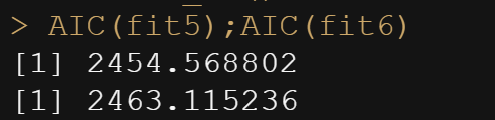AIC is worse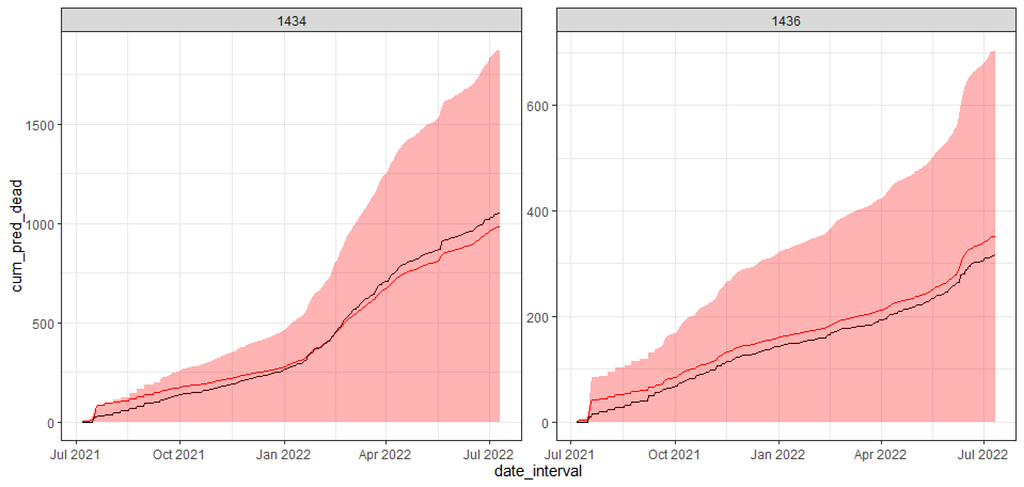But mean prediction is better. Still the confidence intervals are big, just as big as the previous model, but the lines are much closer now.

I will leave it at this for now. I could use the level abstracts for maximum inside temperature and actual outside temperature as well, but then I would also have to do full cross-validation and a rolling time window.

Modeling is not easy and takes a lot of work. What I did was an exercise on how to model mortality and it seems to have some merit, but it sure as hell is not failsafe. That is modeling in a nutshell.

Hope you enjoyed it!

Let me know if something is amis!Modelling Time-To-Event Data in Chickens was originally published in Towards AI on Medium, where people are continuing the conversation by highlighting and responding to this story.

Published via Towards AI